# Madhya Pradesh Board Question Paper for Class 10th Maths 2017 In PDF

## MP Board (MPBSE) Question Paper 2017 Class 10th Mathematics For English Medium with Solutions – Free Download

MP Board Question Paper 2017 Class 10th Maths with solutions can be availed from this page in downloadable pdf format and also in the text, so that learning becomes easier for the students. Maths Question Paper 2017 Class 10 is also added here, which complements the preparation of students within the planned time. Students are able to access all the Madhya Pradesh board previous year maths question papers. The more you practice different types of questions in the previous year papers, the more you succeed in the final board examination.

### MPBSE Class 10th Maths Question Paper With Solutions 2017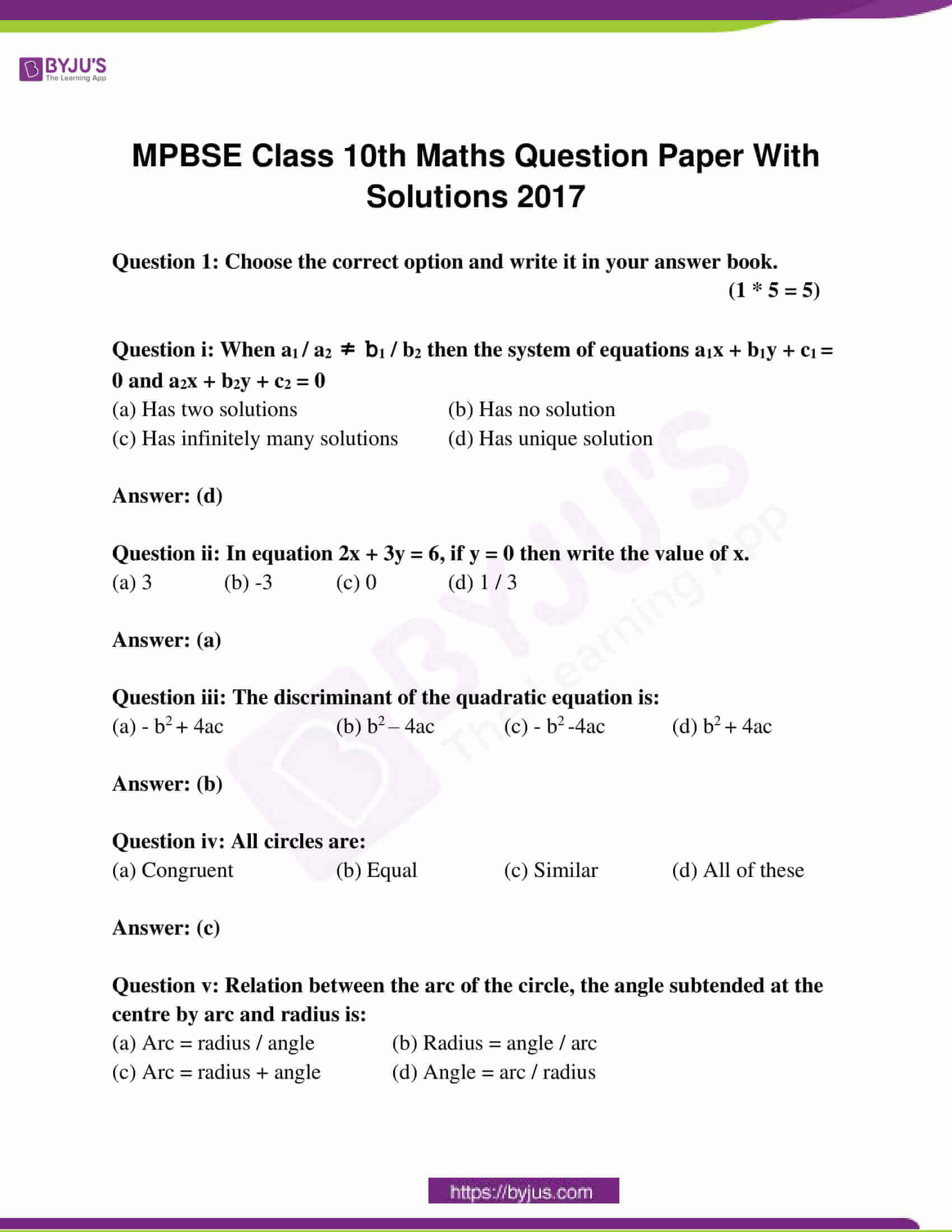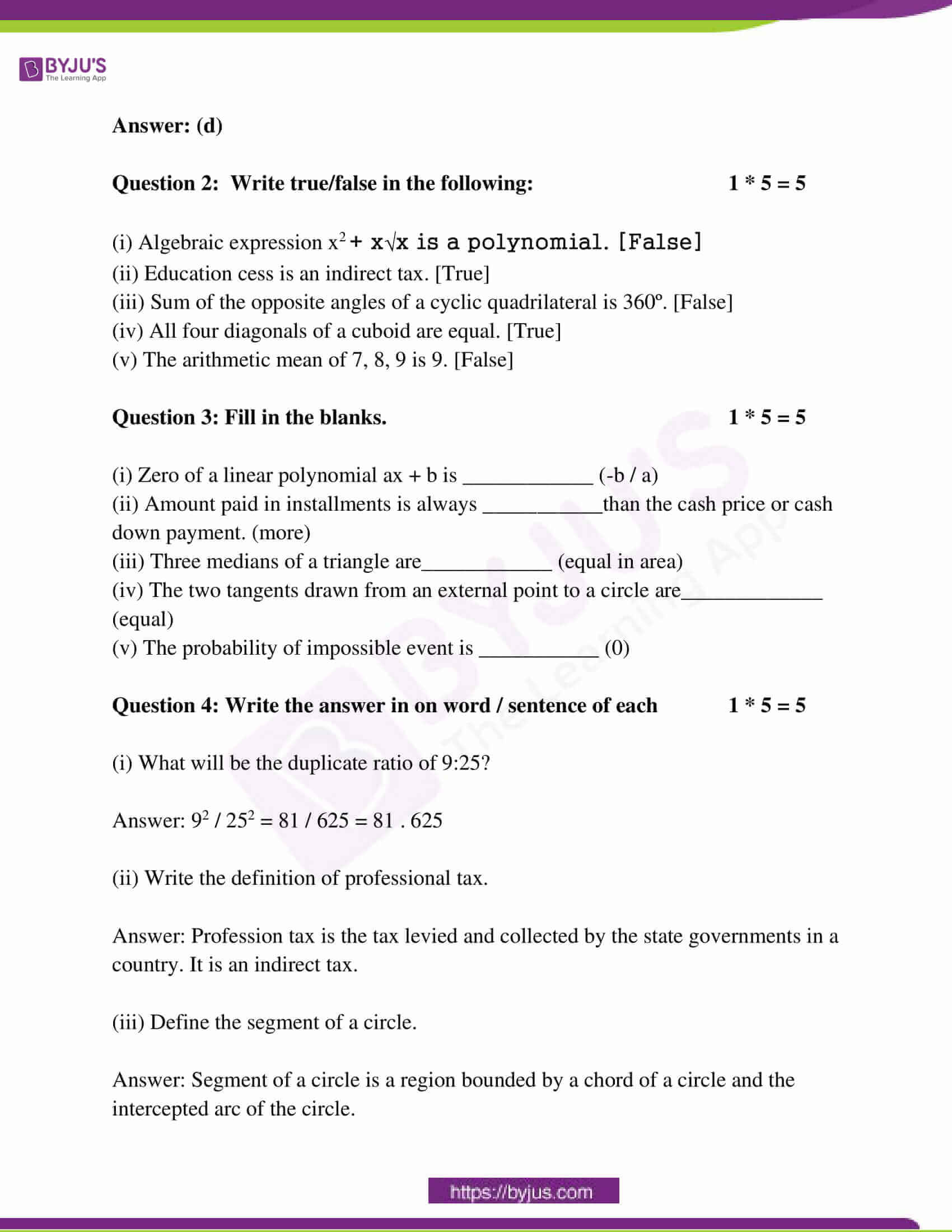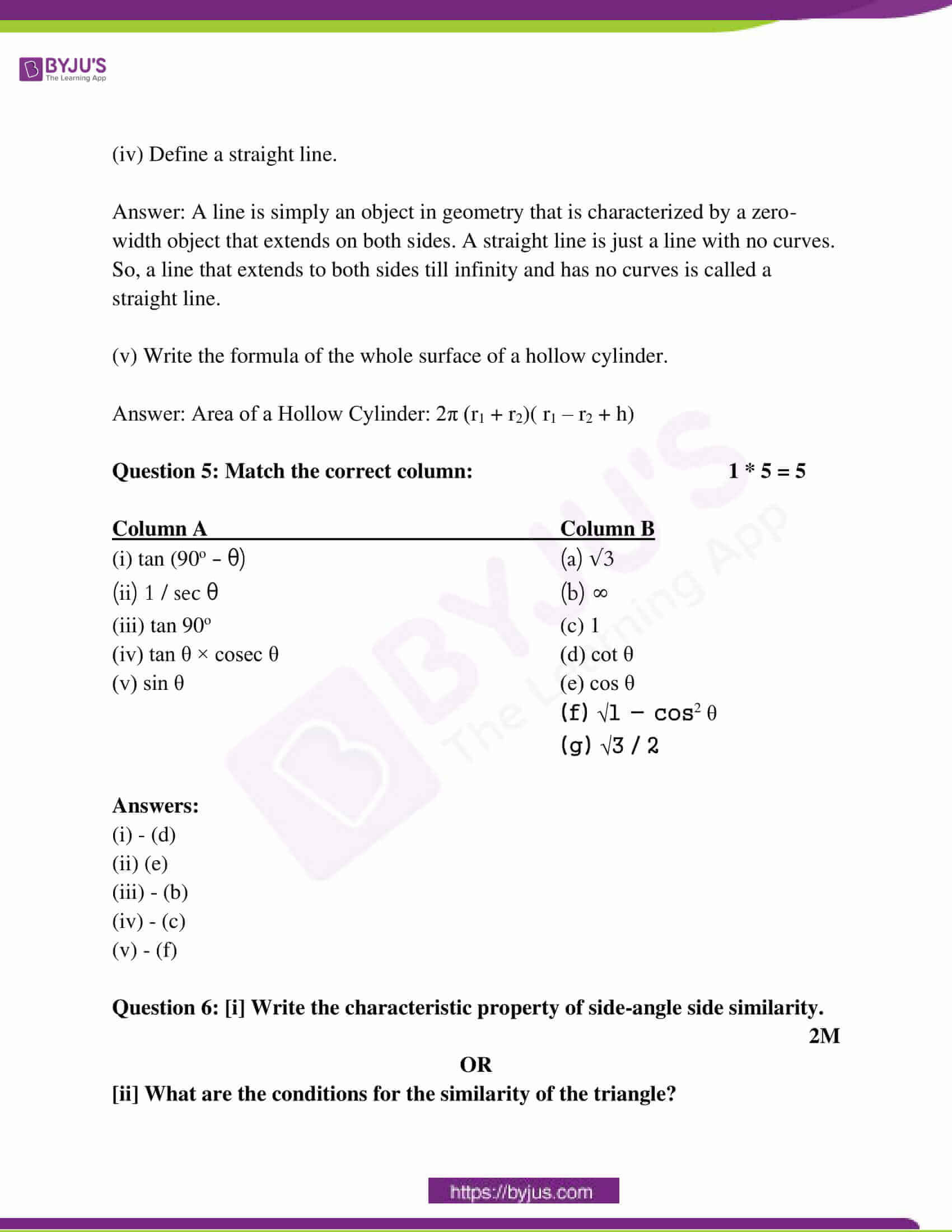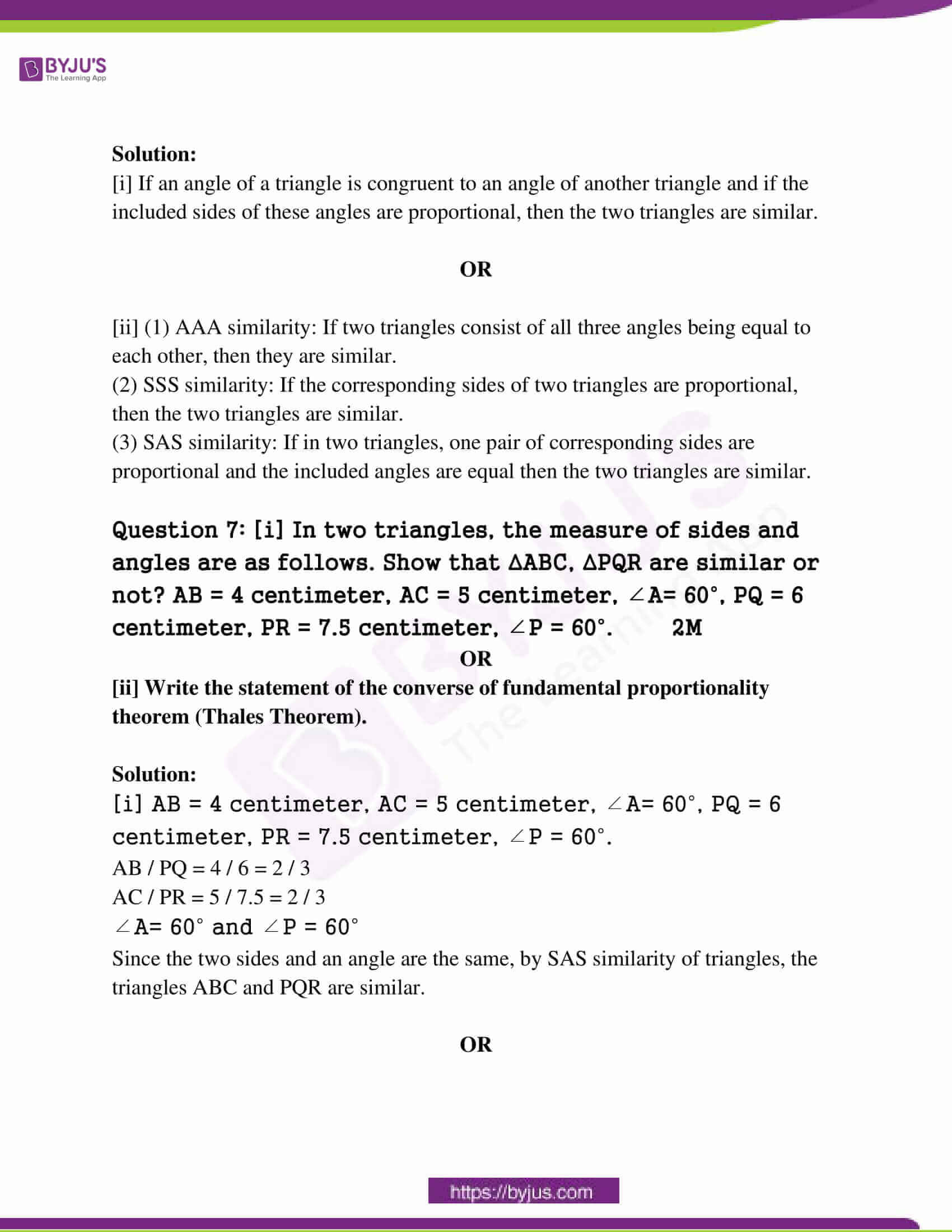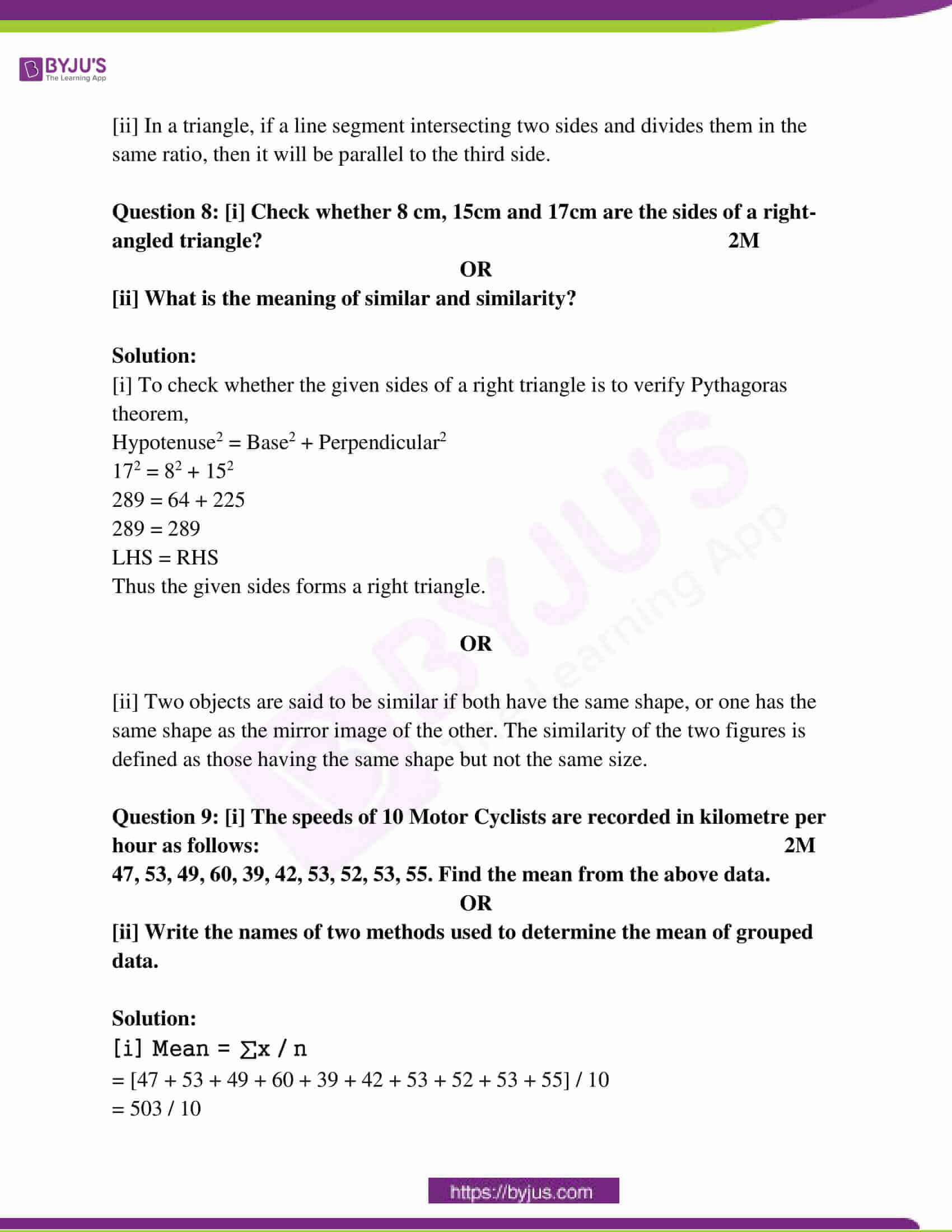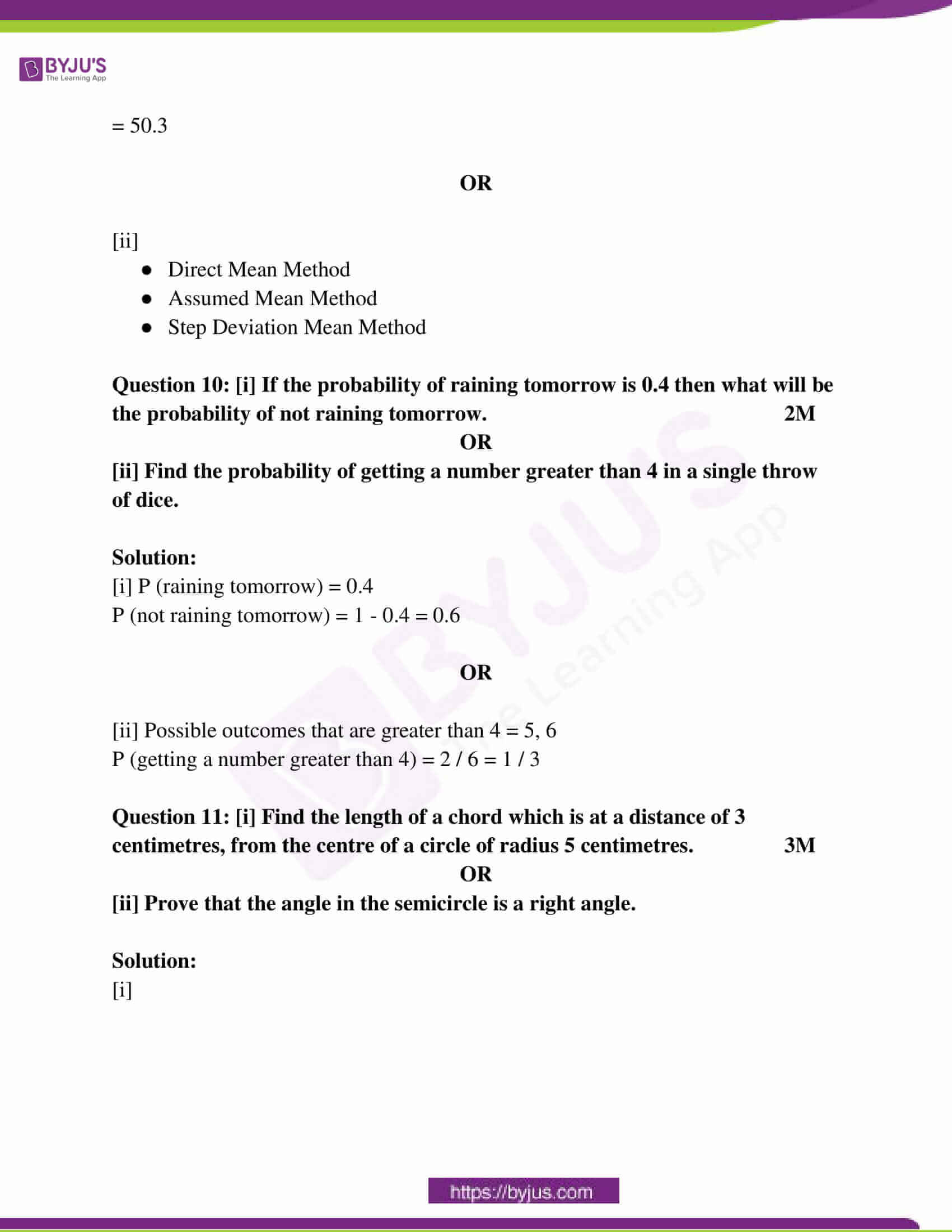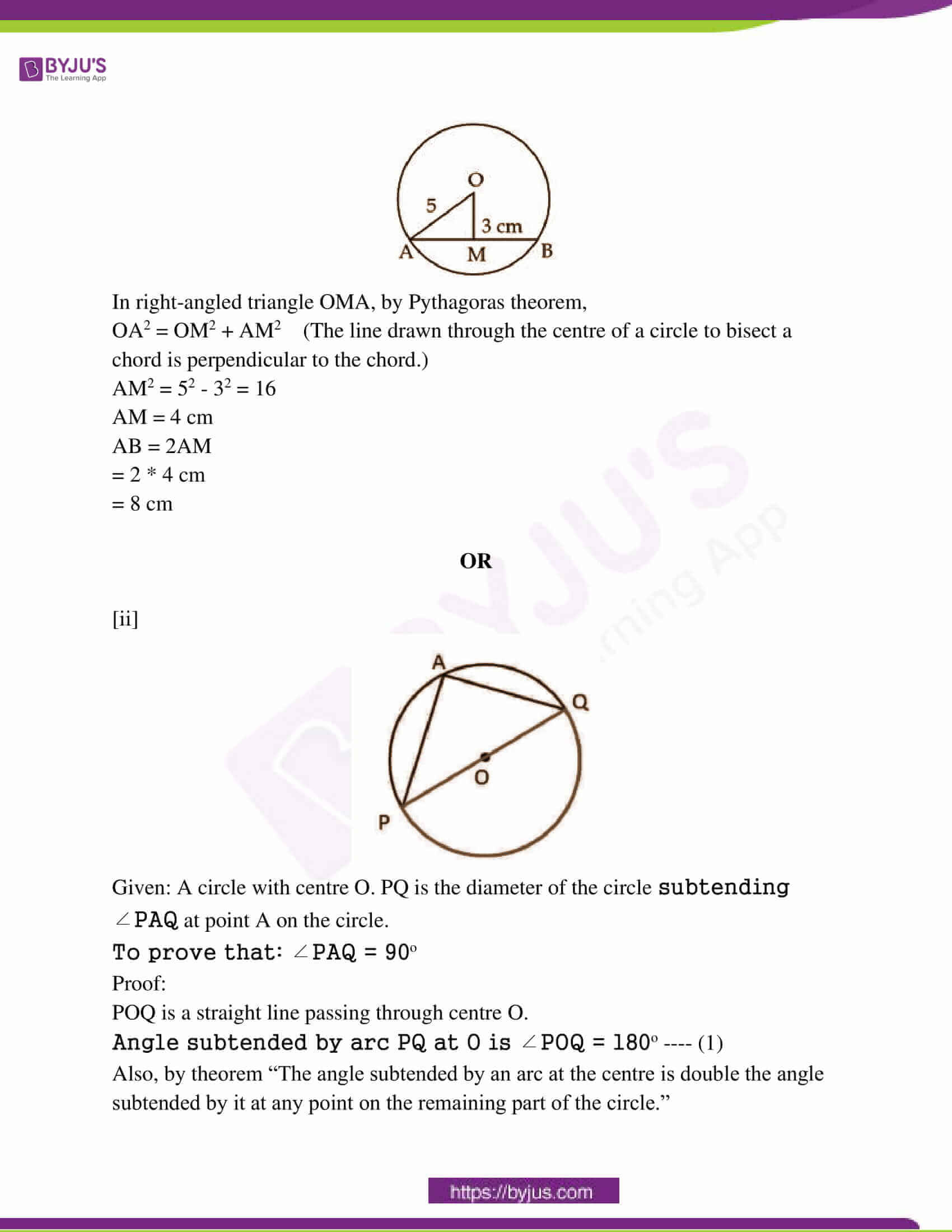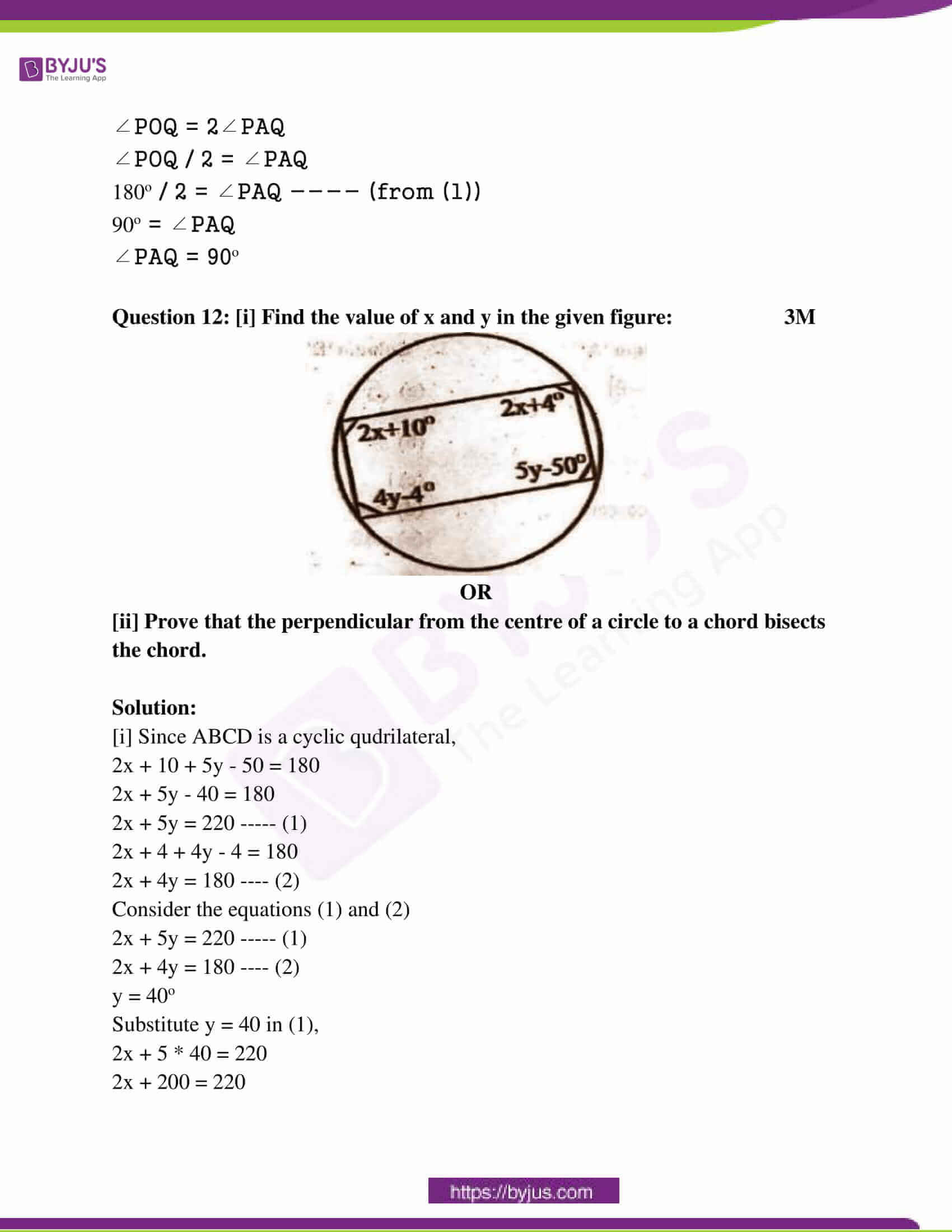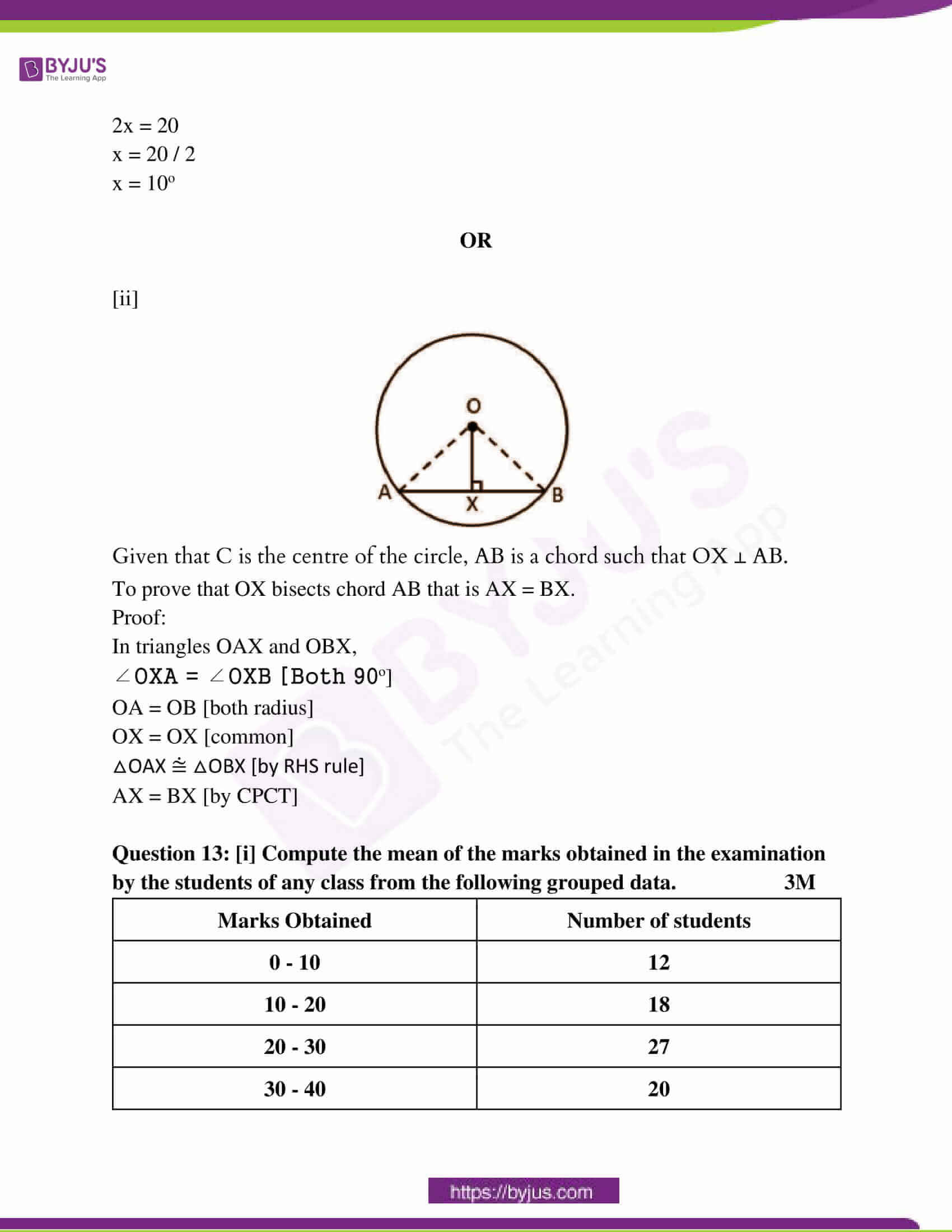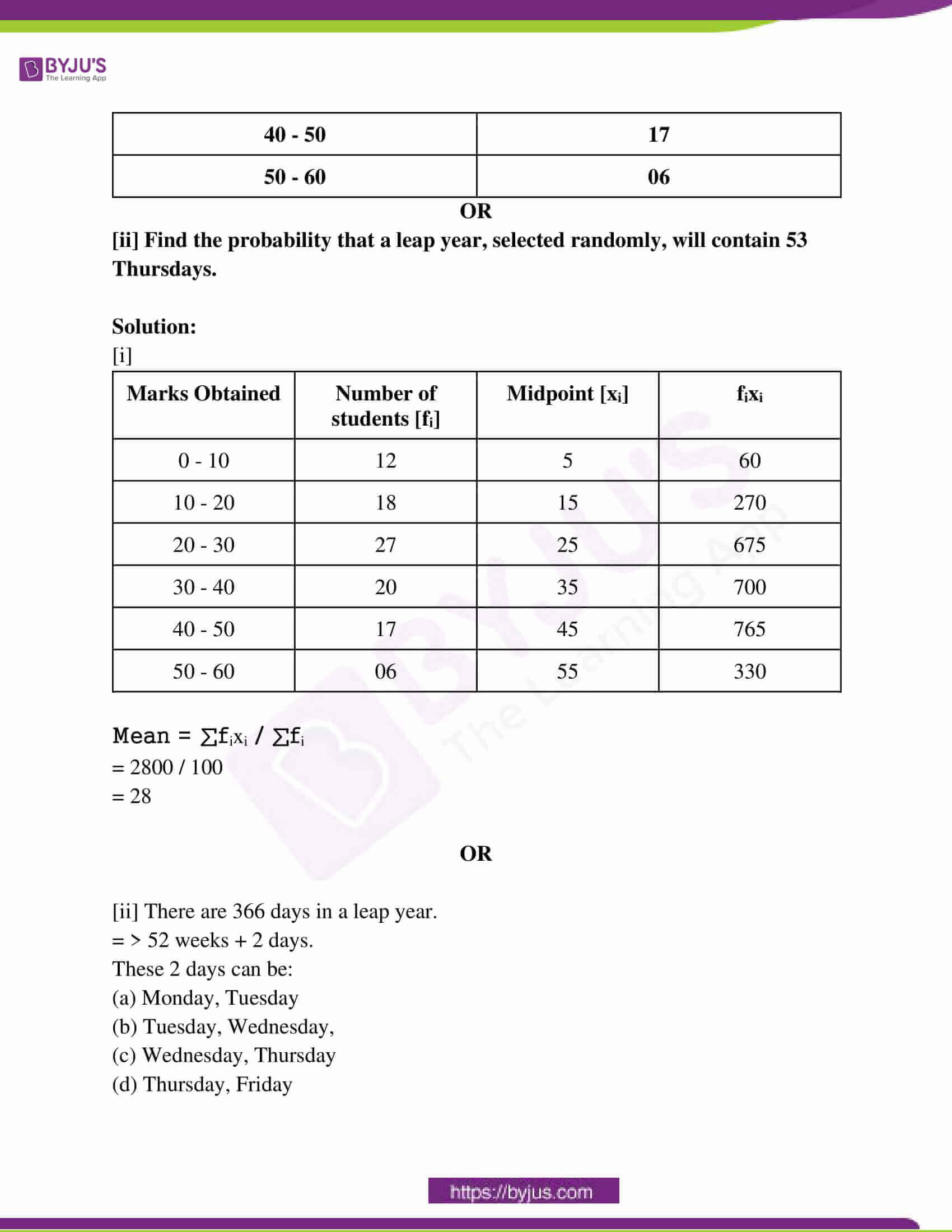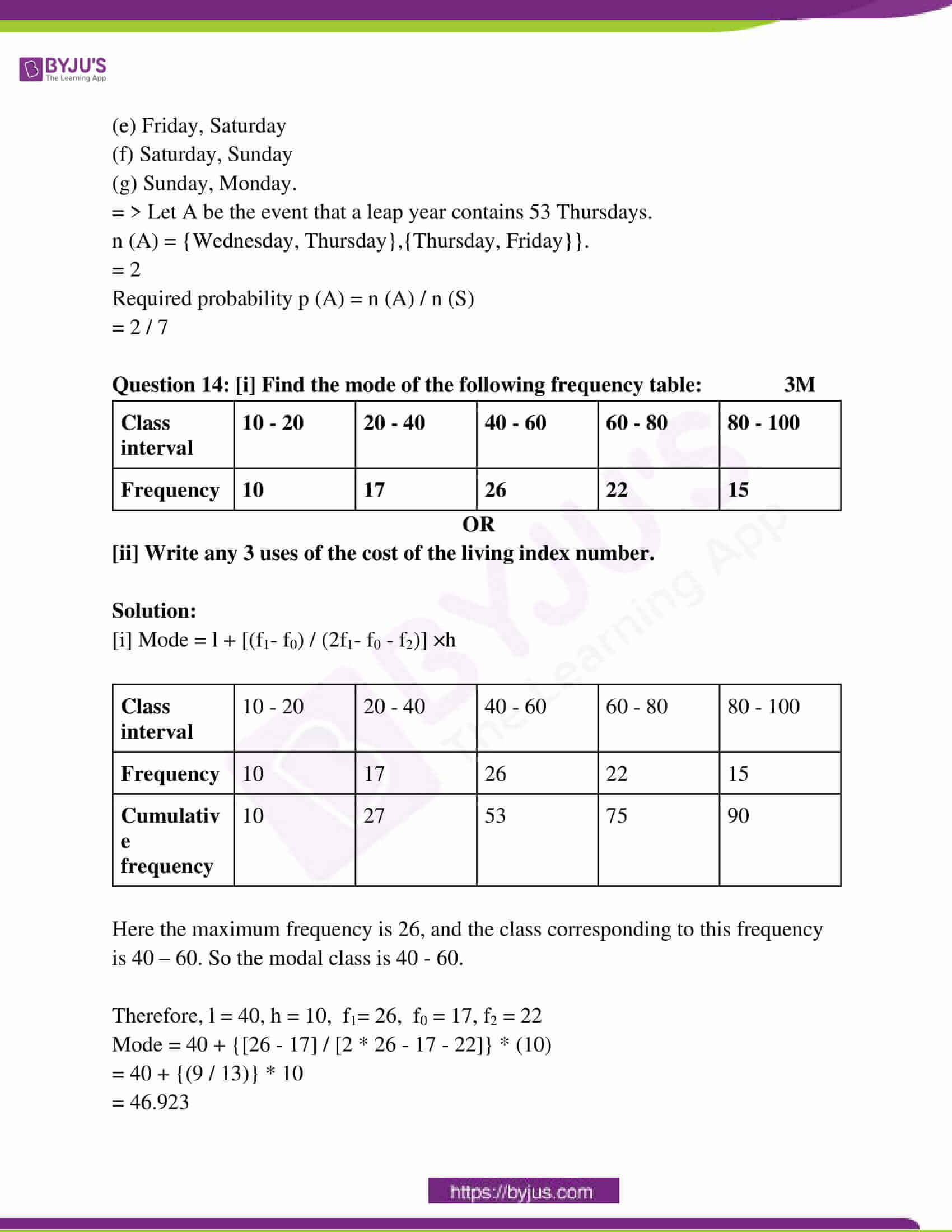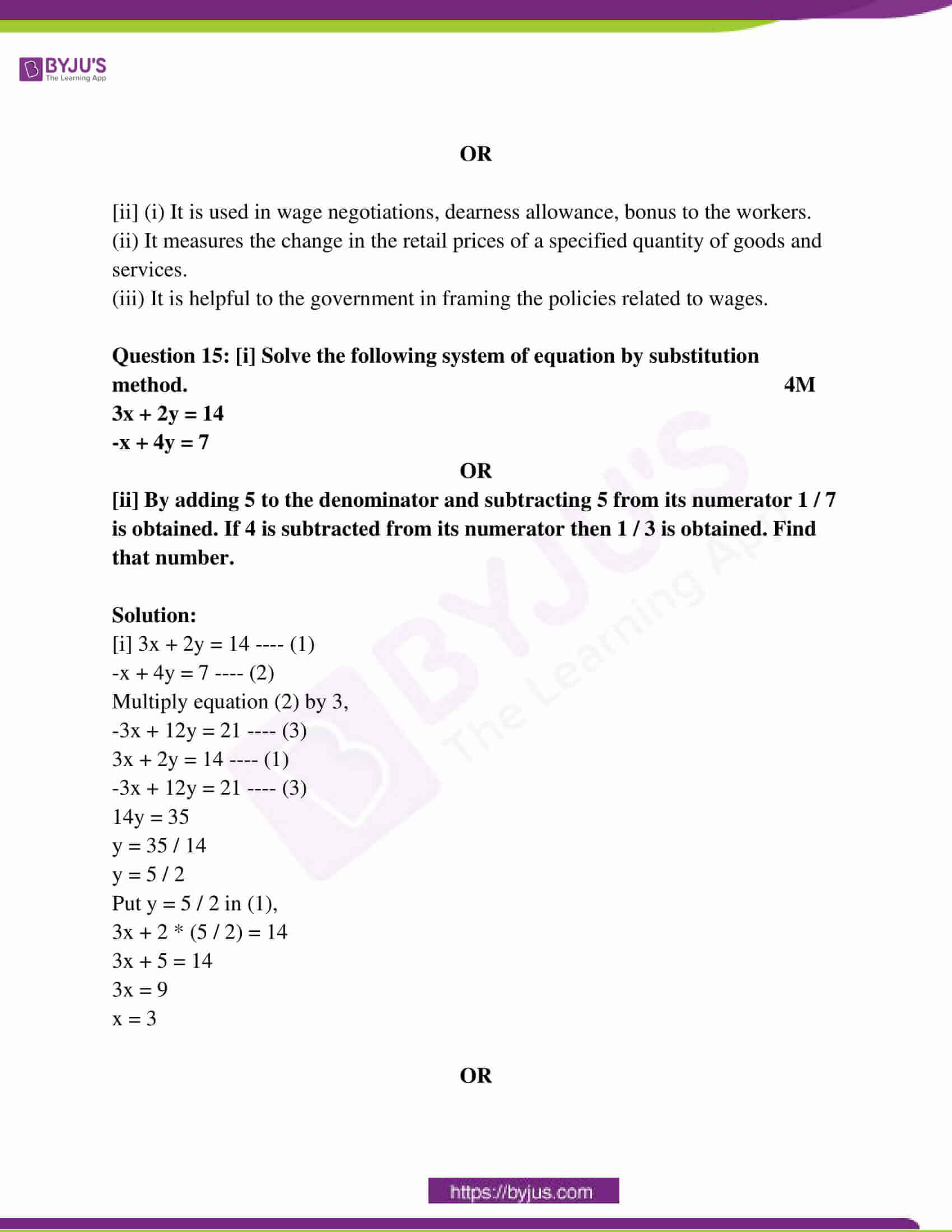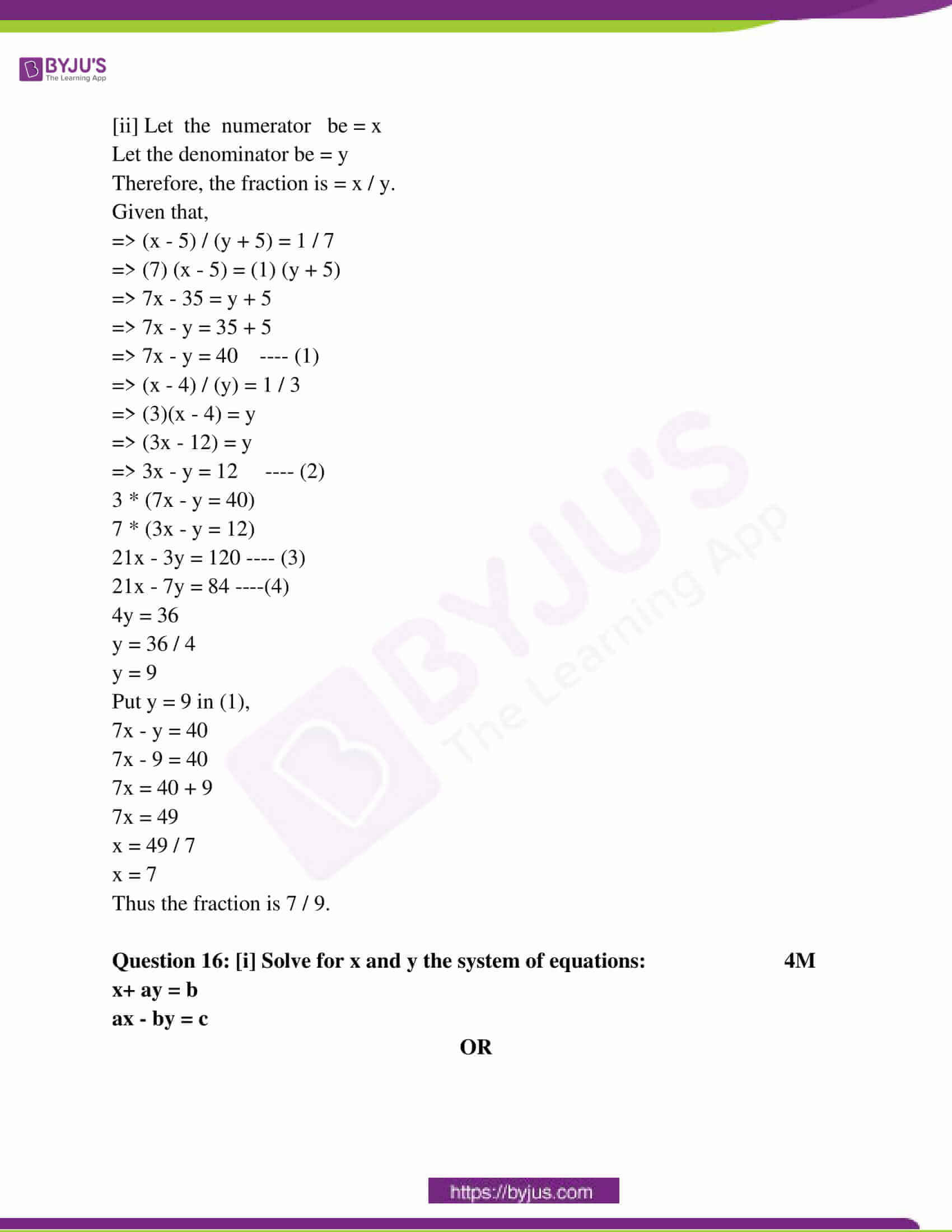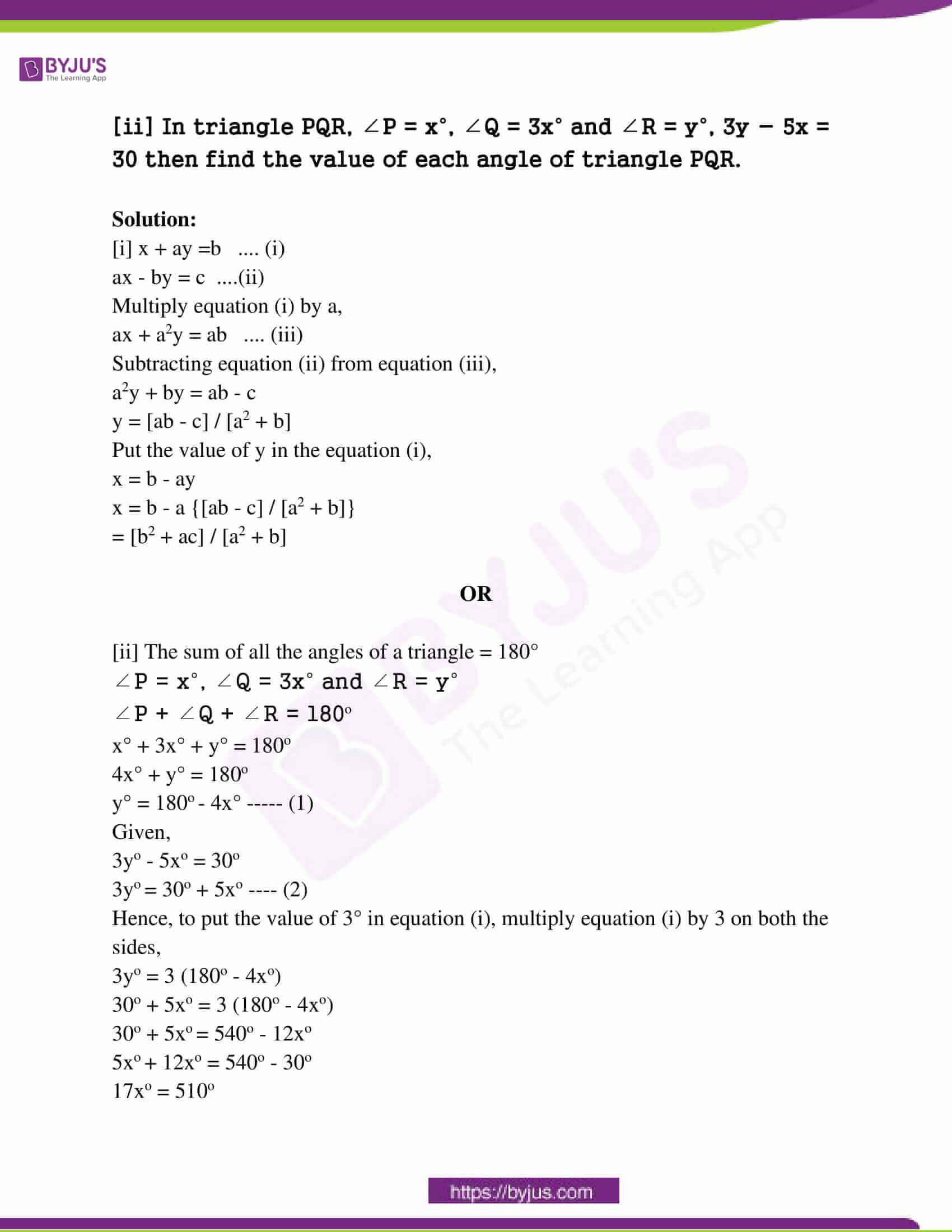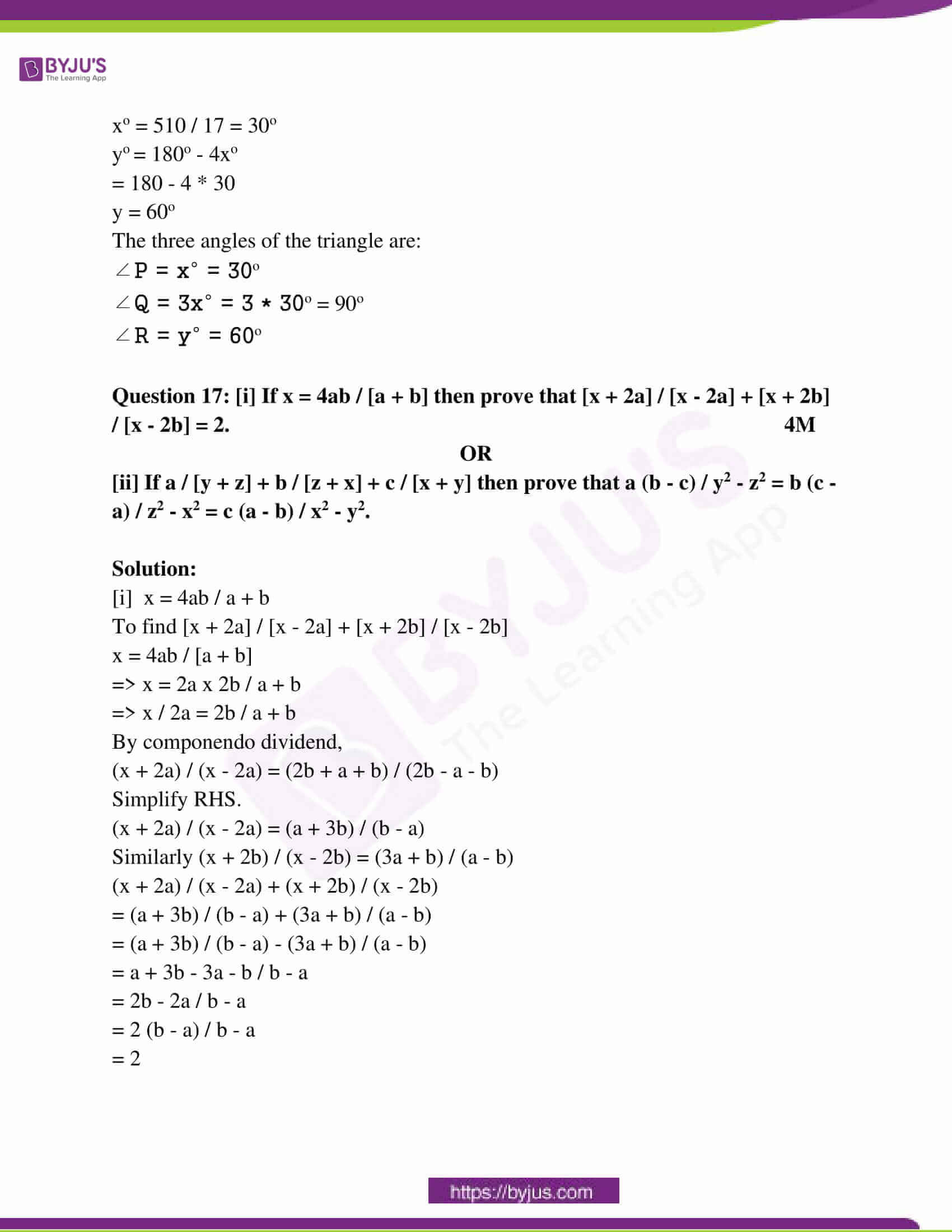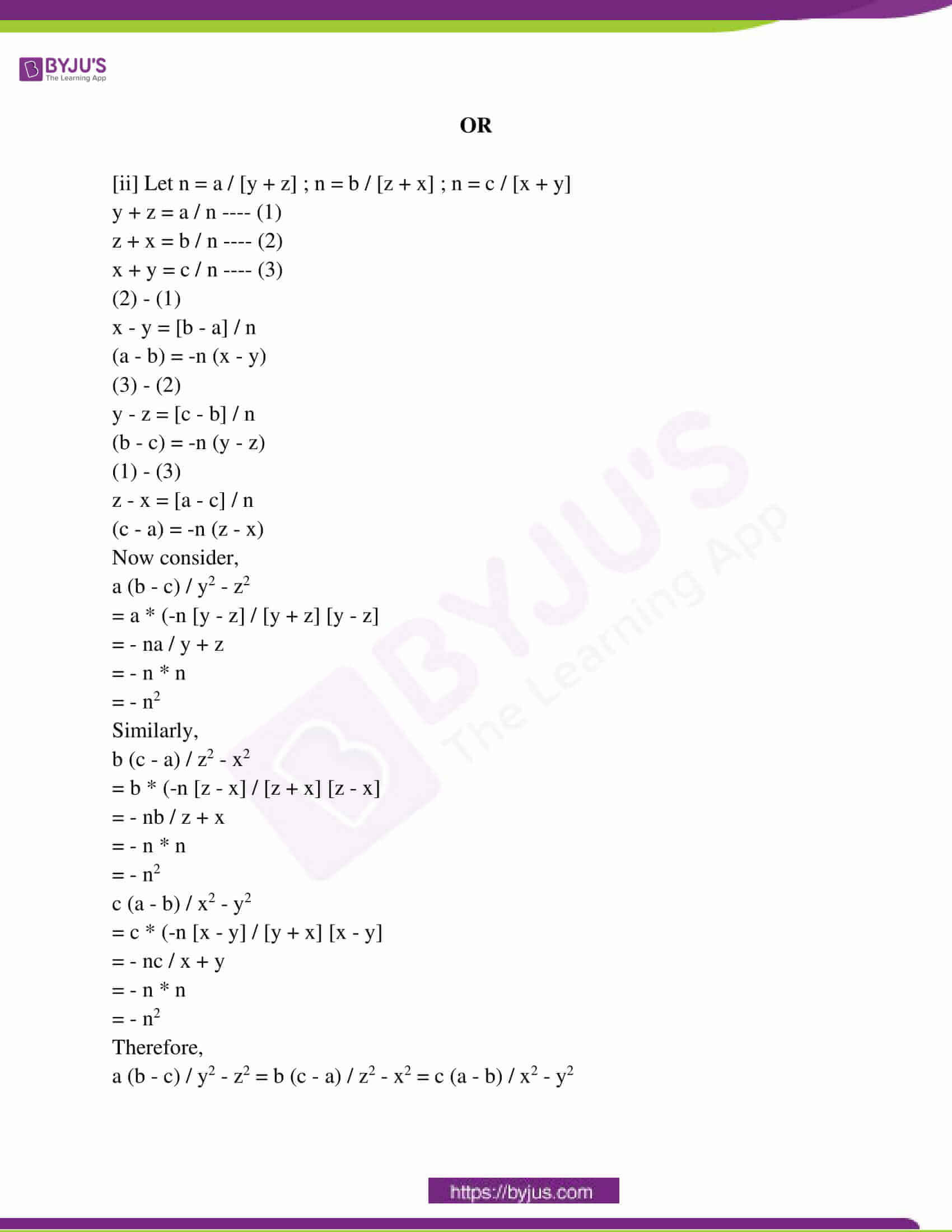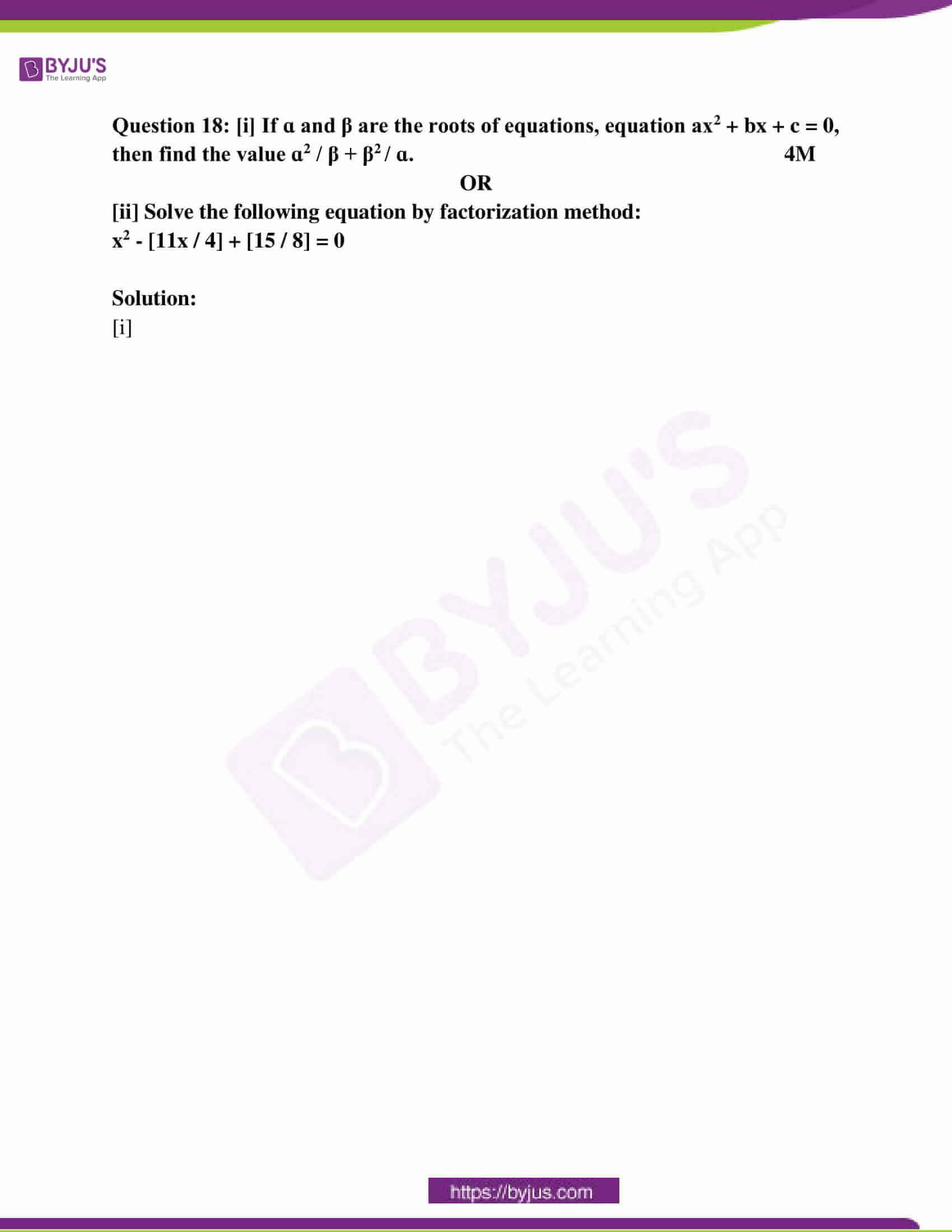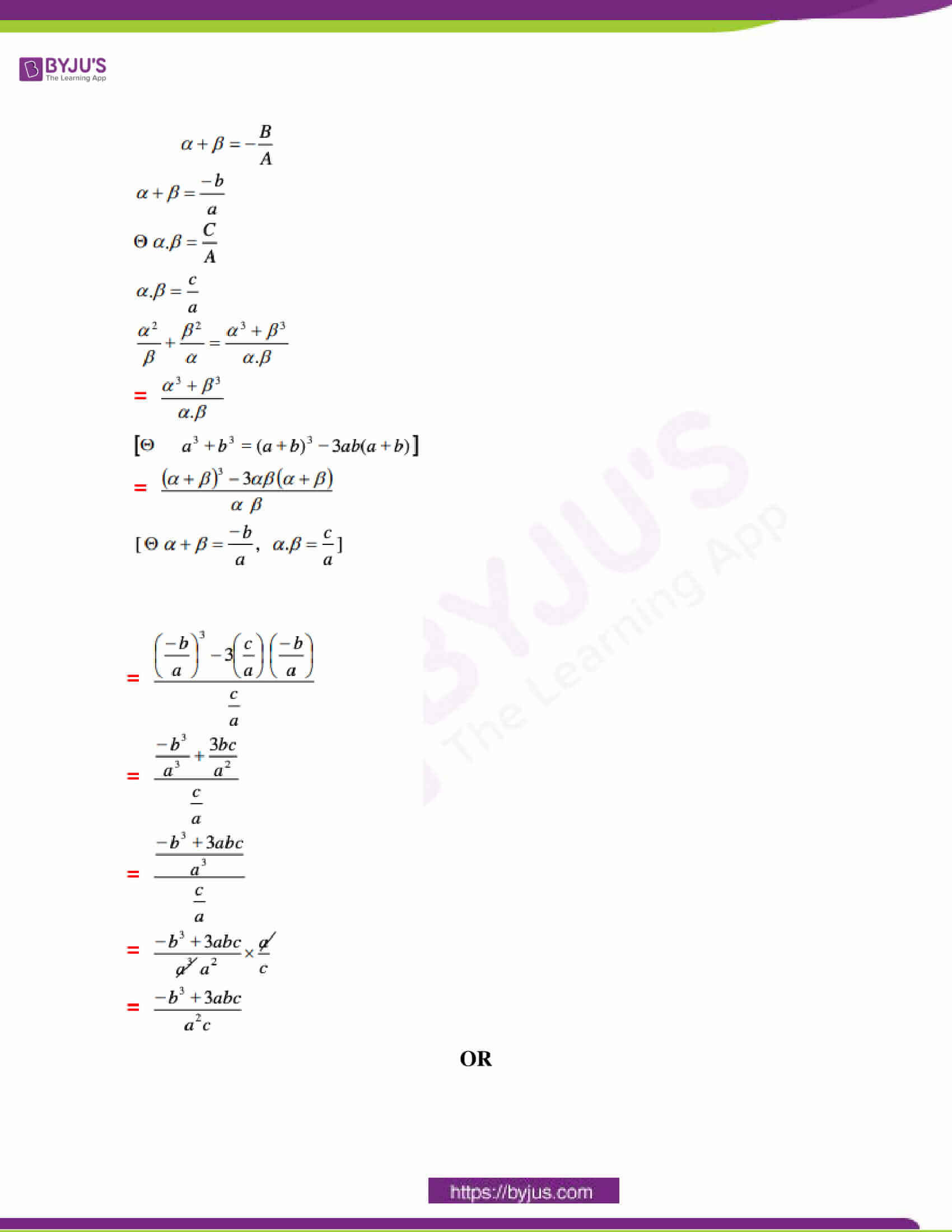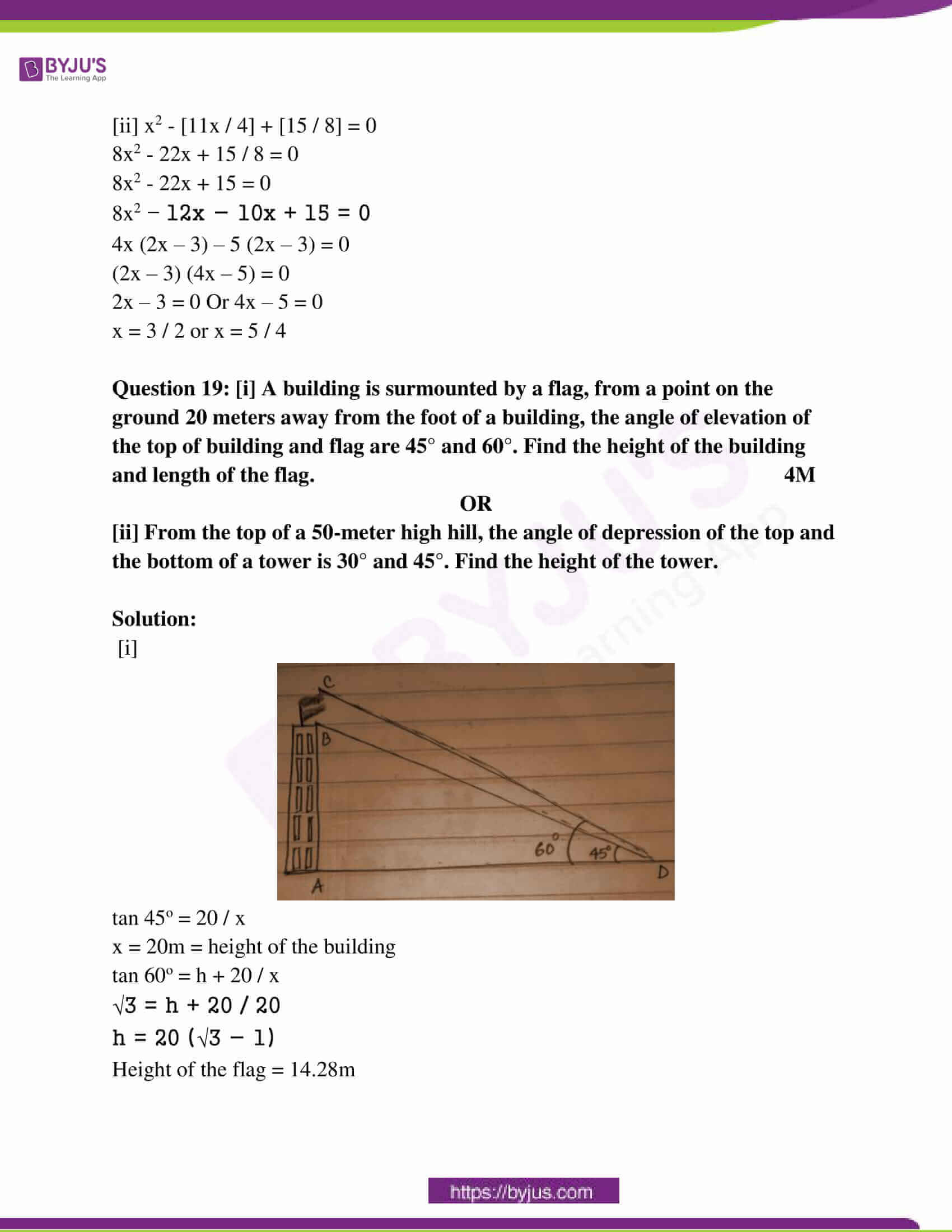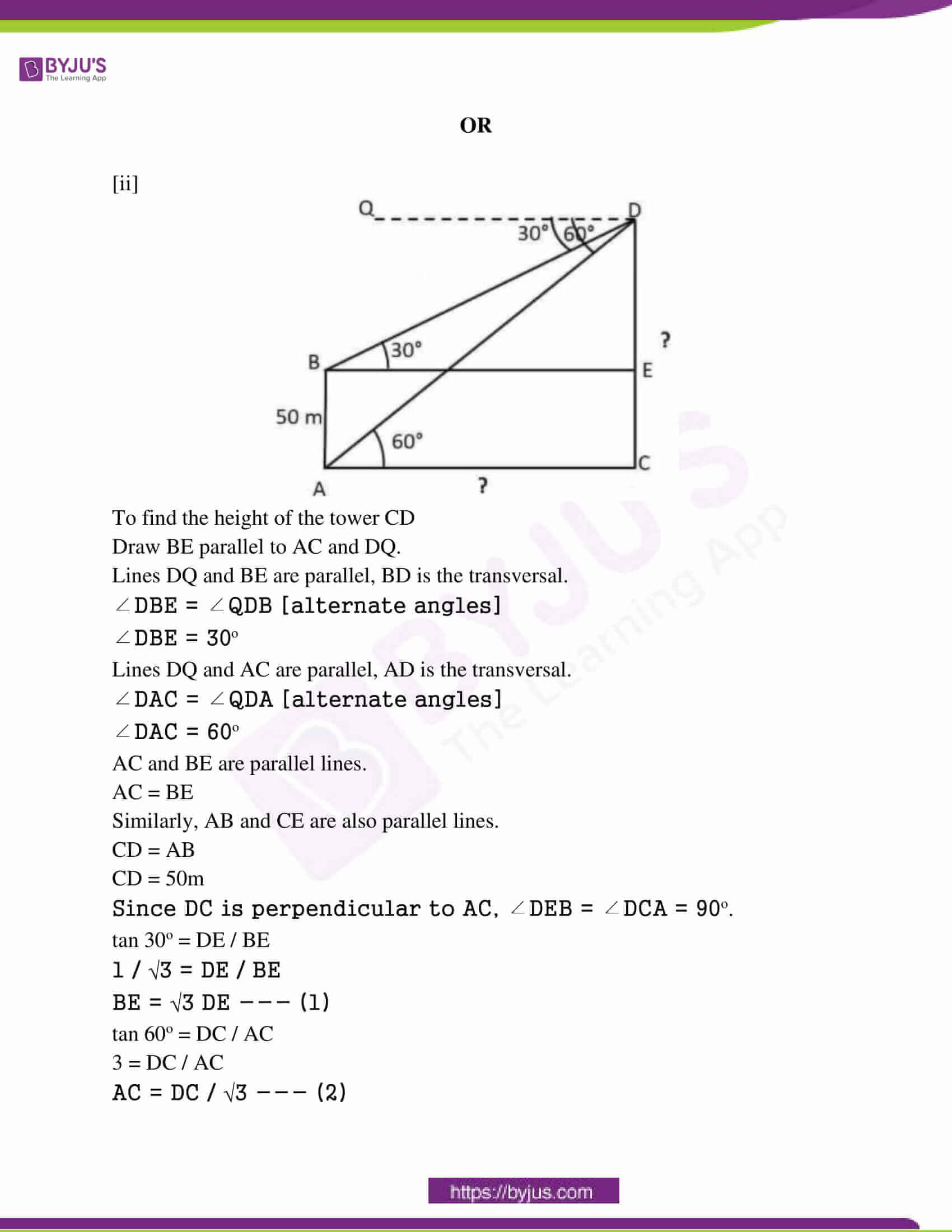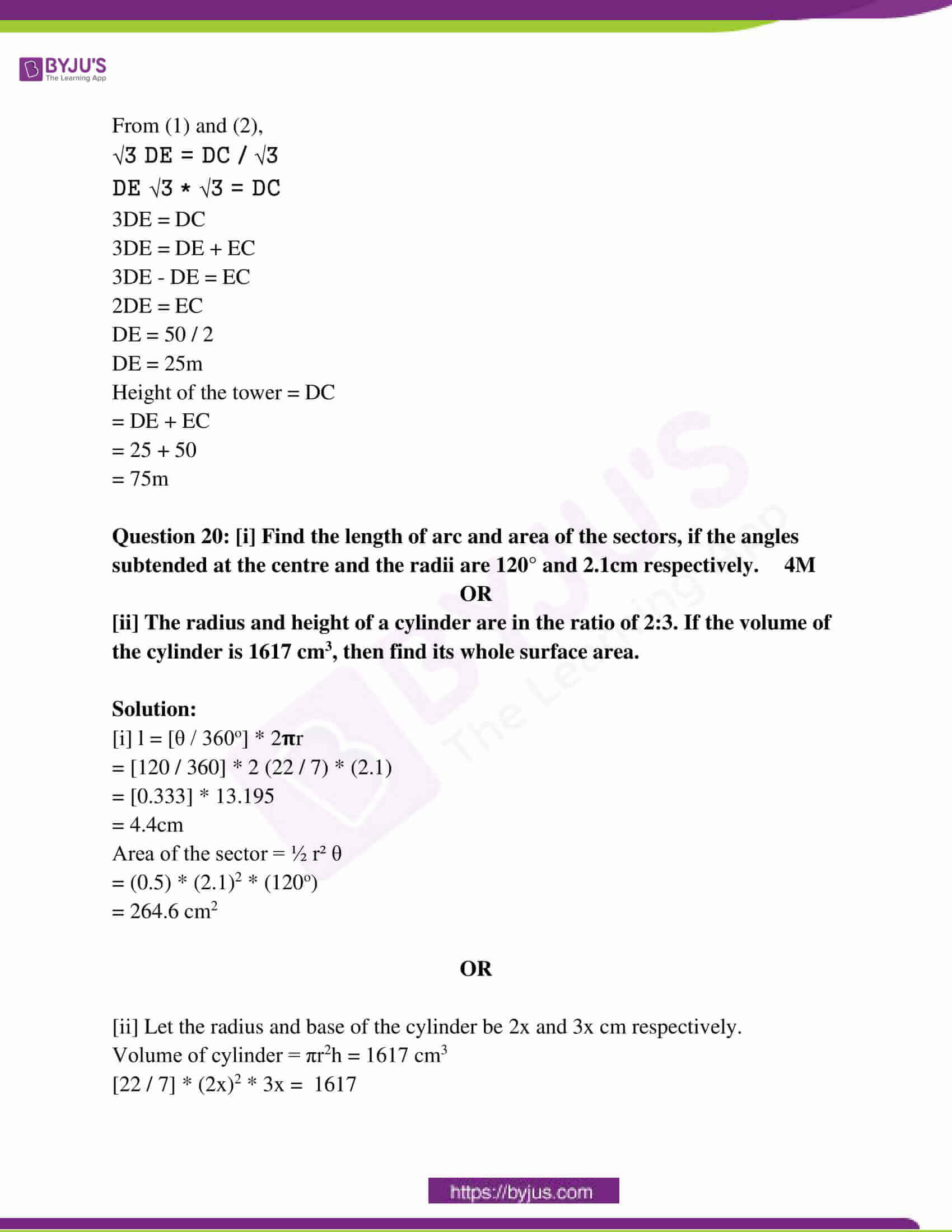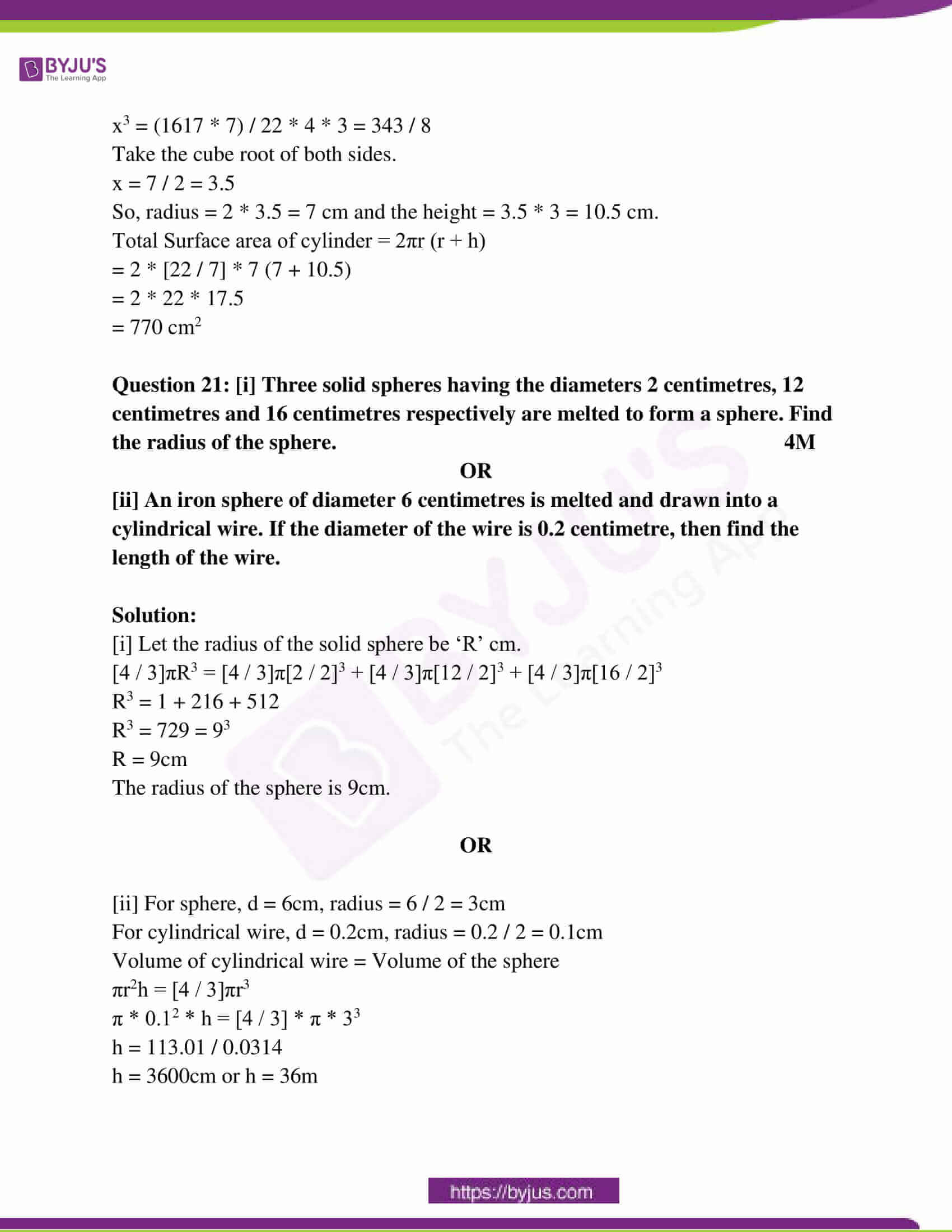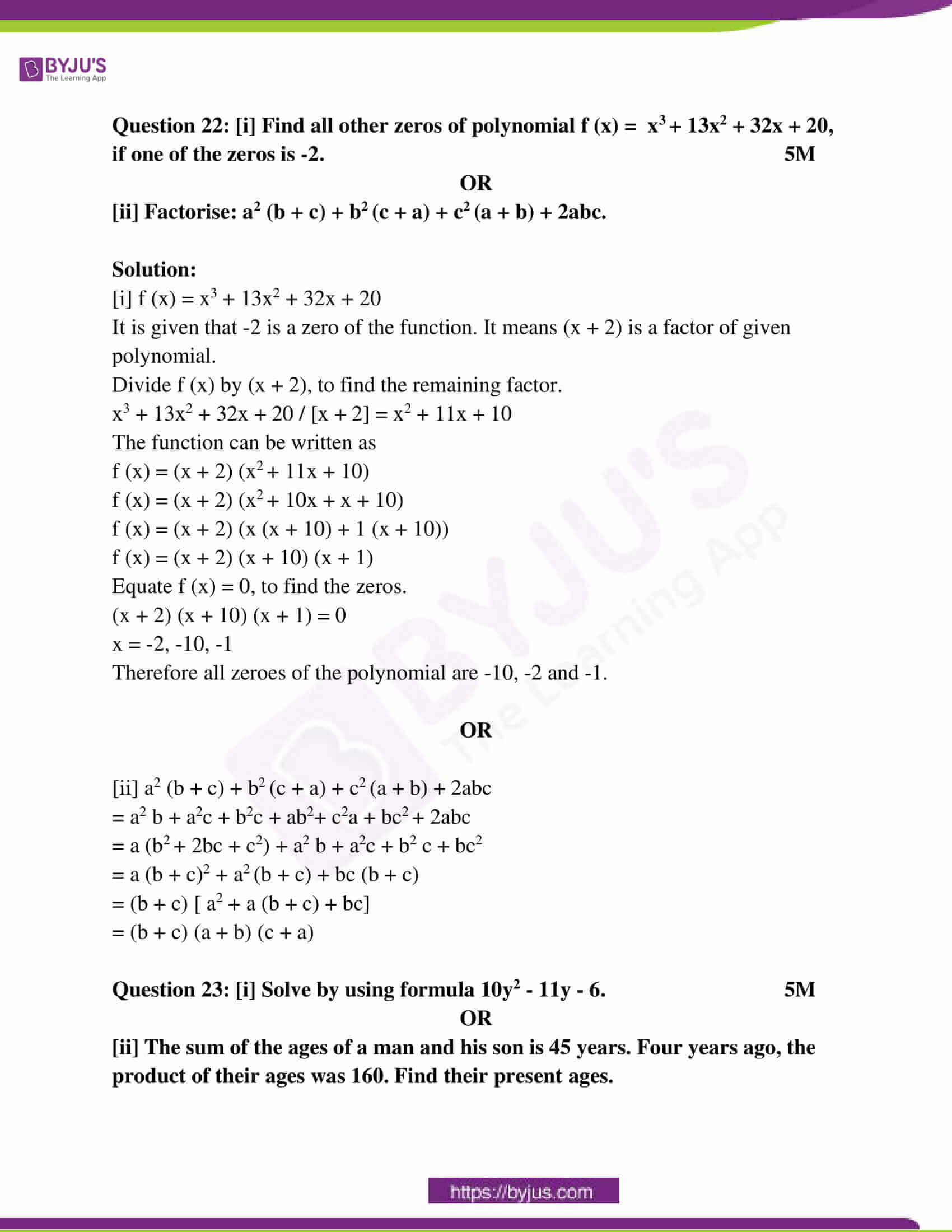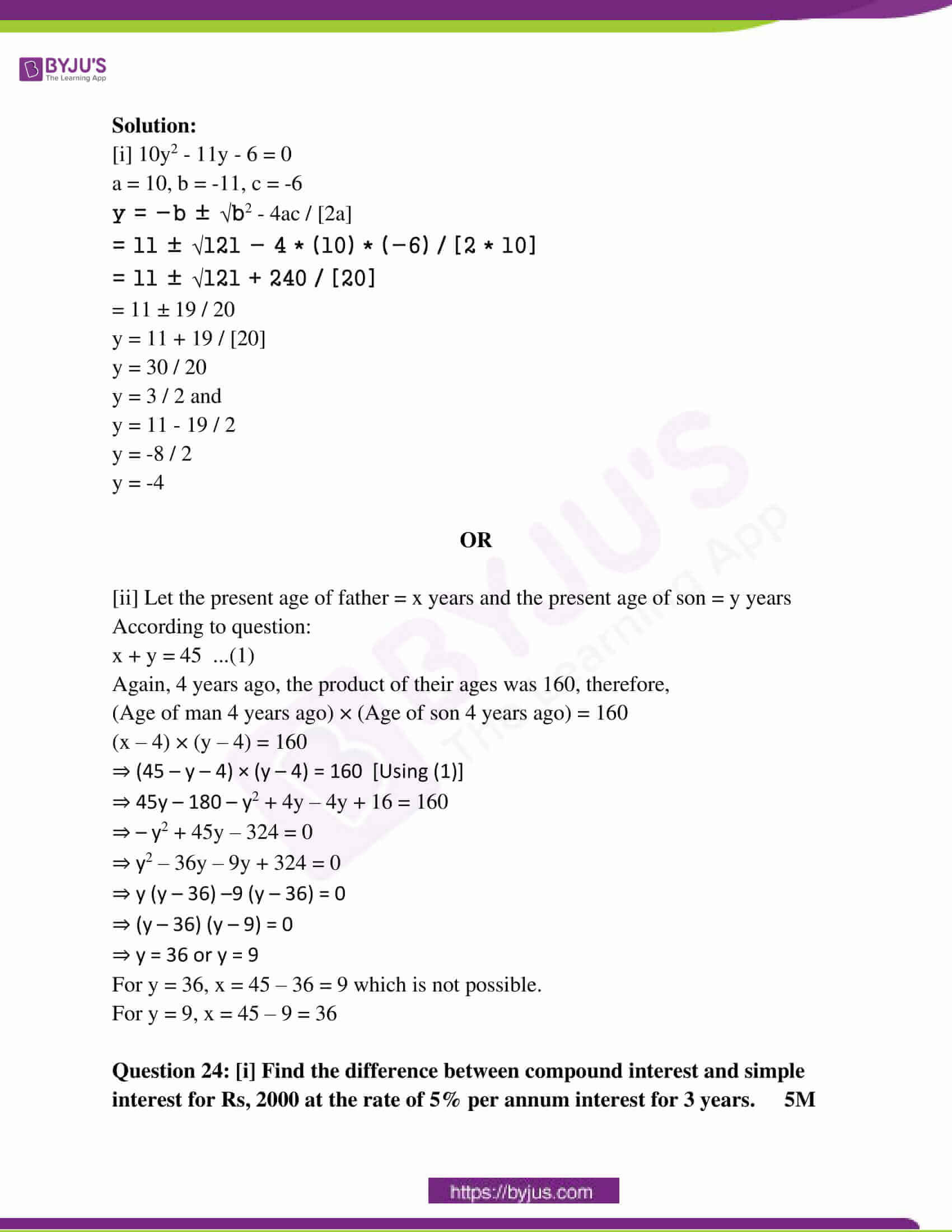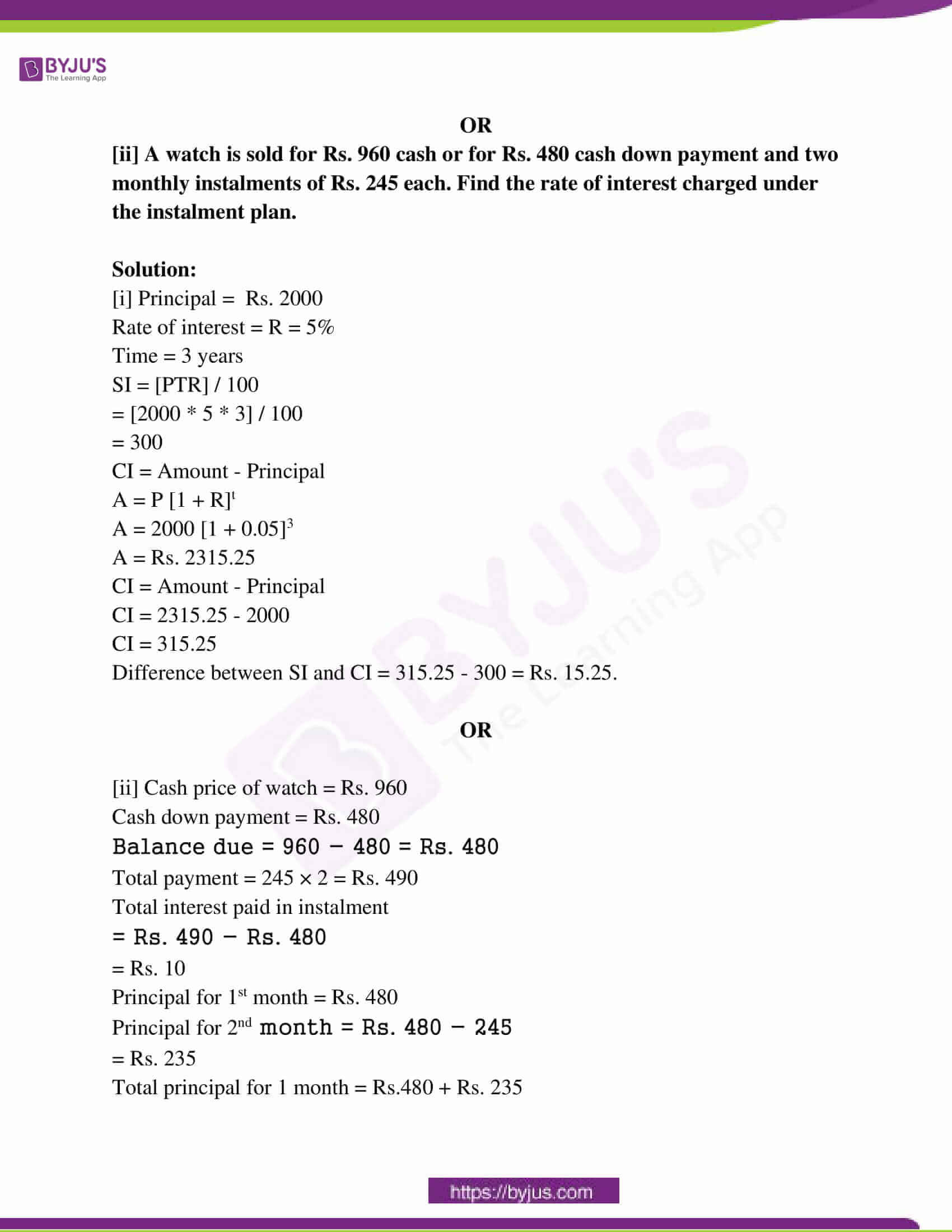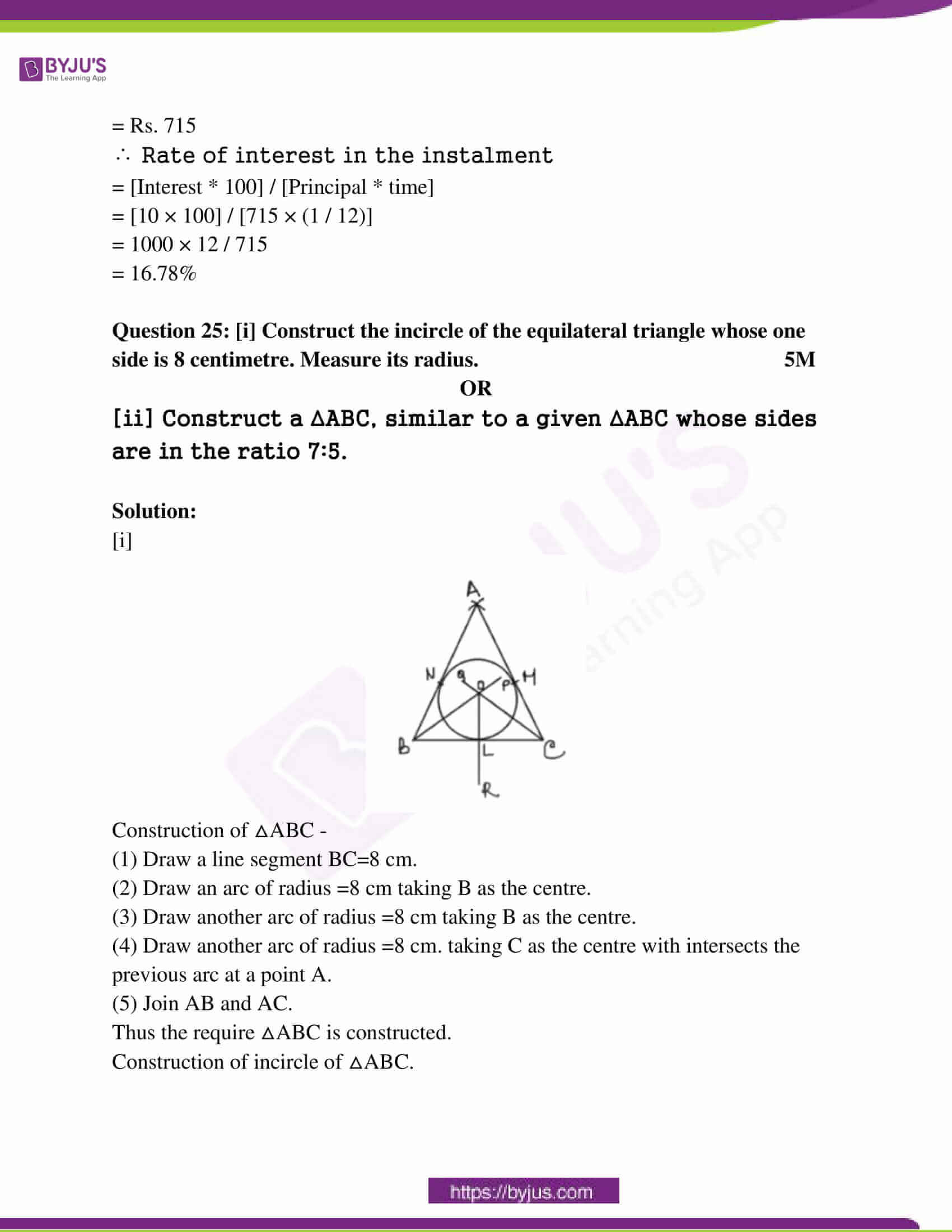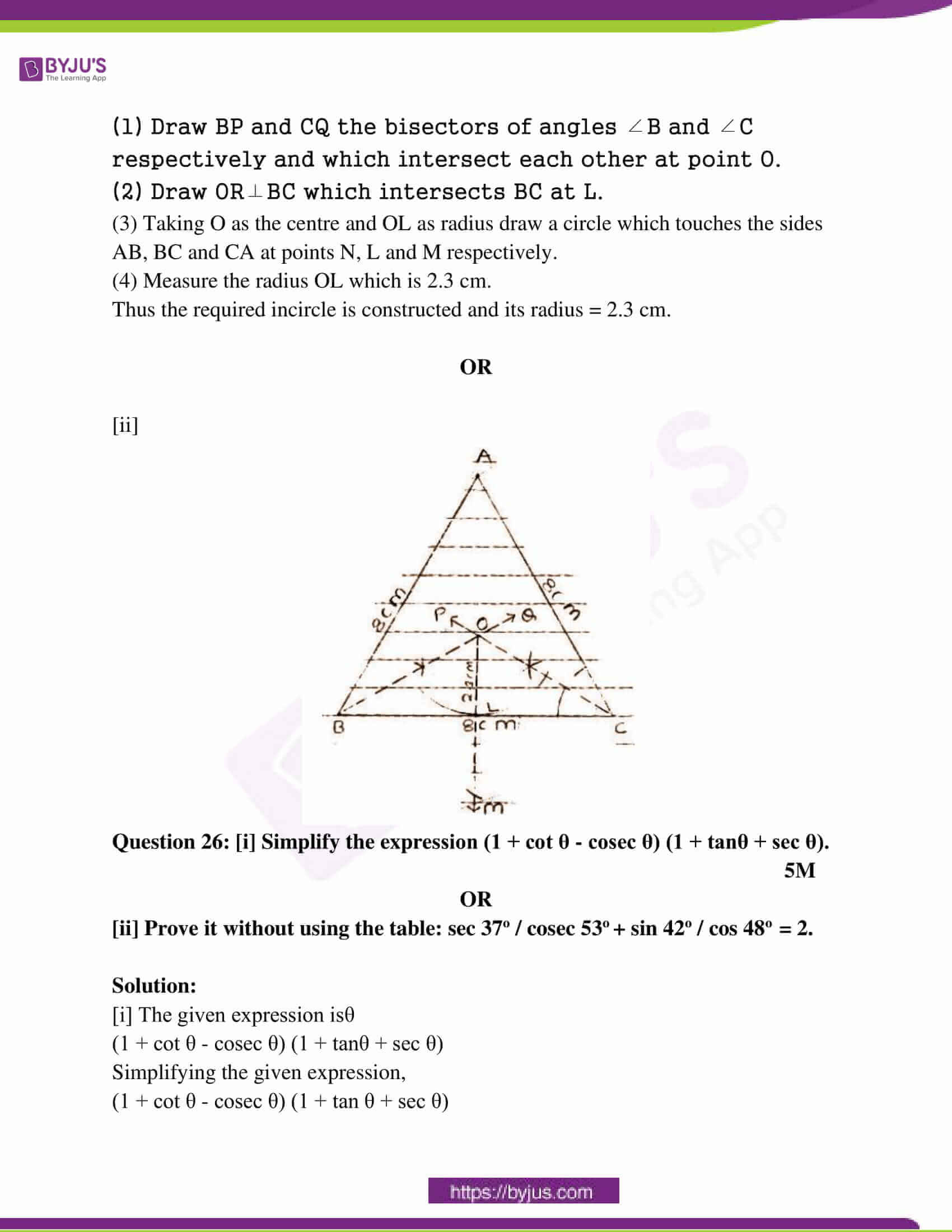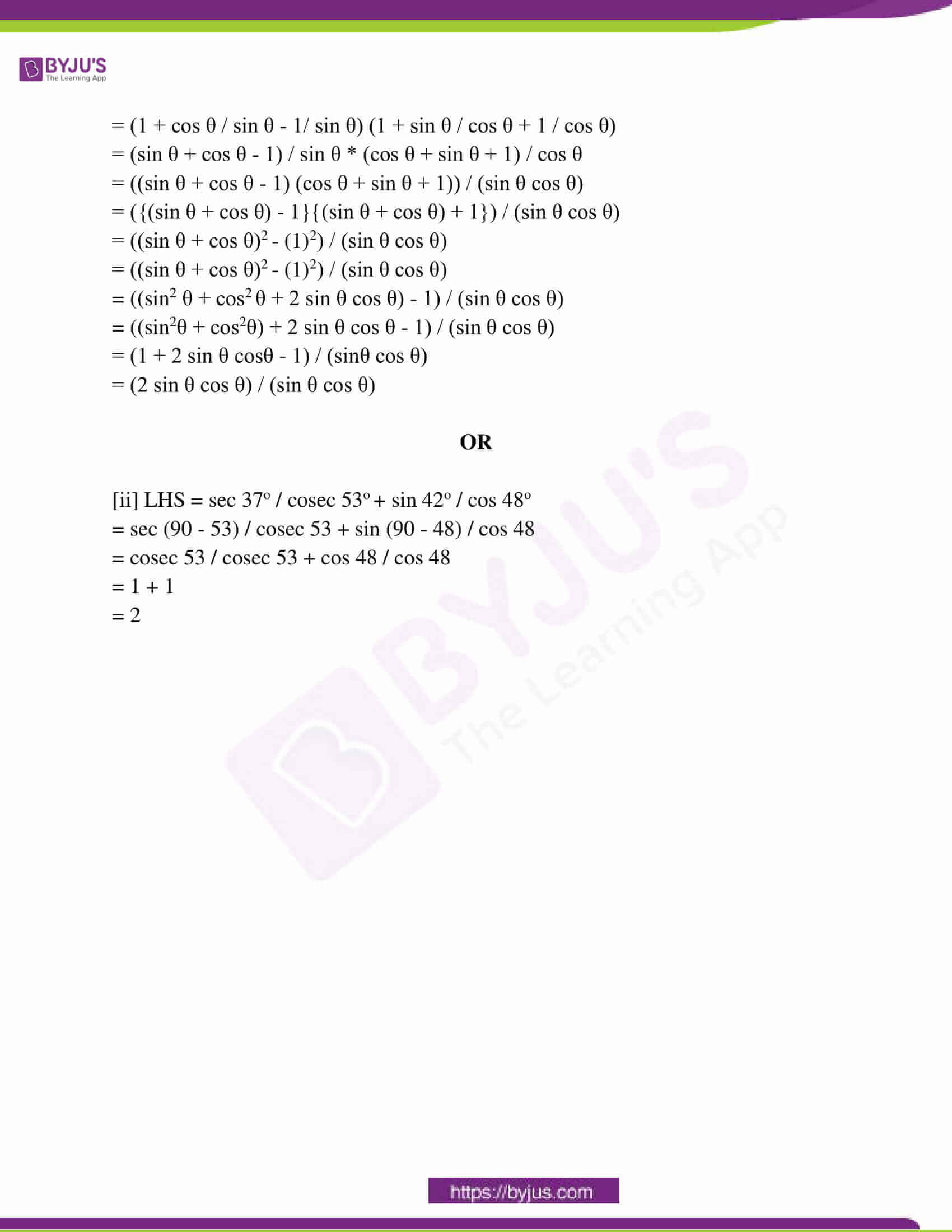Question 1: Choose the correct option and write it in your answer book.

(1 * 5 = 5)

Question i: When a1 / a2 ≠ b1 / b2 then the system of equations a1x + b1y + c1 = 0 and a2x + b2y + c2 = 0

(a) Has two solutions (b) Has no solution

(c) Has infinitely many solutions (d) Has unique solution

Question ii: In equation 2x + 3y = 6, if y = 0 then write the value of x.

(a) 3 (b) -3 (c) 0 (d) 1 / 3

Question iii: The discriminant of the quadratic equation is:

(a) – b2 + 4ac (b) b2 – 4ac (c) – b2 -4ac (d) b2 + 4ac

Question iv: All circles are:

(a) Congruent (b) Equal (c) Similar (d) All of these

Question v: Relation between the arc of the circle, the angle subtended at the centre by arc and radius is:

(a) Arc = radius / angle (b) Radius = angle / arc

(c) Arc = radius + angle (d) Angle = arc / radius

Question 2: Write true/false in the following: 1 * 5 = 5

(i) Algebraic expression x2 + x√x is a polynomial. [False]

(ii) Education cess is an indirect tax. [True]

(iii) Sum of the opposite angles of a cyclic quadrilateral is 360º. [False]

(iv) All four diagonals of a cuboid are equal. [True]

(v) The arithmetic mean of 7, 8, 9 is 9. [False]

Question 3: Fill in the blanks. 1 * 5 = 5

(i) Zero of a linear polynomial ax + b is ____________ (-b / a)

(ii) Amount paid in installments is always ___________than the cash price or cash down payment. (more)

(iii) Three medians of a triangle are____________ (equal in area)

(iv) The two tangents drawn from an external point to a circle are_____________ (equal)

(v) The probability of impossible event is ___________ (0)

Question 4: Write the answer in on word / sentence of each 1 * 5 = 5

(i) What will be the duplicate ratio of 9:25?

Answer: 92 / 252 = 81 / 625 = 81 . 625

(ii) Write the definition of professional tax.

Answer: Profession tax is the tax levied and collected by the state governments in a country. It is an indirect tax.

(iii) Define the segment of a circle.

Answer: Segment of a circle is a region bounded by a chord of a circle and the intercepted arc of the circle.

(iv) Define a straight line.

Answer: A line is simply an object in geometry that is characterized by a zero-width object that extends on both sides. A straight line is just a line with no curves. So, a line that extends to both sides till infinity and has no curves is called a straight line.

(v) Write the formula of the whole surface of a hollow cylinder.

Answer: Area of a Hollow Cylinder: 2π (r1 + r2)( r1 – r2 + h)

Question 5: Match the correct column: 1 * 5 = 5

Column A Column B

(i) tan (90o – θ) (a) √3

(ii) 1 / sec θ (b) ∞

(iii) tan 90o (c) 1

(iv) tan θ × cosec θ (d) cot θ

(v) sin θ (e) cos θ

(f) √1 – cos2 θ

(g) √3 / 2

(i) – (d)

(ii) (e)

(iii) – (b)

(iv) – (c)

(v) – (f)

Question 6: [i] Write the characteristic property of side-angle side similarity.

2M

OR

[ii] What are the conditions for the similarity of the triangle?

Solution:

[i] If an angle of a triangle is congruent to an angle of another triangle and if the included sides of these angles are proportional, then the two triangles are similar.

OR

[ii] (1) AAA similarity: If two triangles consist of all three angles being equal to each other, then they are similar.

(2) SSS similarity: If the corresponding sides of two triangles are proportional, then the two triangles are similar.

(3) SAS similarity: If in two triangles, one pair of corresponding sides are proportional and the included angles are equal then the two triangles are similar.

Question 7: [i] In two triangles, the measure of sides and angles are as follows. Show that ∆ABC, ∆PQR are similar or not? AB = 4 centimeter, AC = 5 centimeter, ∠A= 60°, PQ = 6 centimeter, PR = 7.5 centimeter, ∠P = 60°. 2M

OR

[ii] Write the statement of the converse of fundamental proportionality theorem (Thales Theorem).

Solution:

[i] AB = 4 centimeter, AC = 5 centimeter, ∠A= 60°, PQ = 6 centimeter, PR = 7.5 centimeter, ∠P = 60°.

AB / PQ = 4 / 6 = 2 / 3

AC / PR = 5 / 7.5 = 2 / 3

∠A= 60° and ∠P = 60°

Since the two sides and an angle are the same, by SAS similarity of triangles, the triangles ABC and PQR are similar.

OR

[ii] In a triangle, if a line segment intersects two sides and divides them in the same ratio, then it will be parallel to the third side.

Question 8: [i] Check whether 8 cm, 15cm and 17cm are the sides of a right-angled triangle? 2M

OR

[ii] What is the meaning of similar and similarity?

Solution:

[i] To check whether the given sides of a right triangle is to verify Pythagoras theorem,

Hypotenuse2 = Base2 + Perpendicular2

172 = 82 + 152

289 = 64 + 225

289 = 289

LHS = RHS

Thus the given sides form a right triangle.

OR

[ii] Two objects are said to be similar if both have the same shape, or one has the same shape as the mirror image of the other. The similarity of the two figures is defined as those having the same shape but not the same size.

Question 9: [i] The speeds of 10 Motor Cyclists are recorded in kilometre per hour as follows: 2M

47, 53, 49, 60, 39, 42, 53, 52, 53, 55. Find the mean from the above data.

OR

[ii] Write the names of two methods used to determine the mean of grouped data.

Solution:

[i] Mean = ∑x / n

= [47 + 53 + 49 + 60 + 39 + 42 + 53 + 52 + 53 + 55] / 10

= 503 / 10

= 50.3

OR

[ii]
• Direct Mean Method
• Assumed Mean Method
• Step Deviation Mean Method

Question 10: [i] If the probability of raining tomorrow is 0.4 then what will be the probability of not raining tomorrow. 2M

OR

[ii] Find the probability of getting a number greater than 4 in a single throw of dice.

Solution:

[i] P (raining tomorrow) = 0.4

P (not raining tomorrow) = 1 – 0.4 = 0.6

OR

[ii] Possible outcomes that are greater than 4 = 5, 6

P (getting a number greater than 4) = 2 / 6 = 1 / 3

Question 11: [i] Find the length of a chord which is at a distance of 3 centimetres, from the centre of a circle of radius 5 centimetres. 3M

OR

[ii] Prove that the angle in the semicircle is a right angle.

Solution:

[i]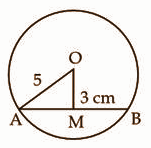In right-angled triangle OMA, by Pythagoras theorem,

OA2 = OM2 + AM2 (The line drawn through the centre of a circle to bisect a chord is perpendicular to the chord.)

AM2 = 52 – 32 = 16

AM = 4 cm

AB = 2AM

= 2 * 4 cm

= 8 cm

OR

[ii]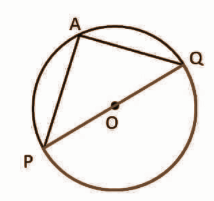Given: A circle with centre O. PQ is the diameter of the circle subtending ∠PAQ at point A on the circle.

To prove that: ∠PAQ = 90o

Proof:

POQ is a straight line passing through centre O.

Angle subtended by arc PQ at O is ∠POQ = 180o —- (1)

Also, by theorem “The angle subtended by an arc at the centre is double the angle subtended by it at any point on the remaining part of the circle.”

∠POQ = 2∠PAQ

∠POQ / 2 = ∠PAQ

180o / 2 = ∠PAQ —- (from (1))

90o = ∠PAQ

∠PAQ = 90o

Question 12: [i] Find the value of x and y in the given figure: 3M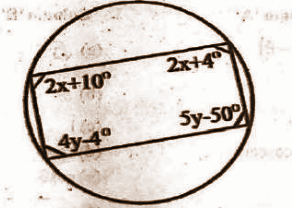OR

[ii] Prove that the perpendicular from the centre of a circle to a chord bisects the chord.

Solution:

[i] Since ABCD is a cyclic quadrilateral,

2x + 10 + 5y – 50 = 180

2x + 5y – 40 = 180

2x + 5y = 220 —– (1)

2x + 4 + 4y – 4 = 180

2x + 4y = 180 —- (2)

Consider the equations (1) and (2)

2x + 5y = 220 —– (1)

2x + 4y = 180 —- (2)

y = 40o

Substitute y = 40 in (1),

2x + 5 * 40 = 220

2x + 200 = 220

2x = 20

x = 20 / 2

x = 10o

OR

[ii]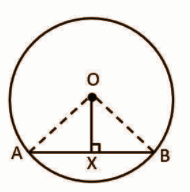Given that C is the centre of the circle, AB is a chord such that OX ⟂ AB.

To prove that OX bisects chord AB that is AX = BX.

Proof:

In triangles OAX and OBX,

∠OXA = ∠OXB [Both 90o]

OX = OX [common]

△OAX ⩭ △OBX [by RHS rule]

AX = BX [by CPCT]

Question 13: [i] Compute the mean of the marks obtained in the examination by the students of any class from the following grouped data. 3M

 Marks Obtained Number of students 0 – 10 12 10 – 20 18 20 – 30 27 30 – 40 20 40 – 50 17 50 – 60 06

OR

[ii] Find the probability that a leap year, selected randomly, will contain 53 Thursdays.

Solution:

[i]
 Marks Obtained Number of students [fi] Midpoint [xi] fixi 0 – 10 12 5 60 10 – 20 18 15 270 20 – 30 27 25 675 30 – 40 20 35 700 40 – 50 17 45 765 50 – 60 06 55 330

Mean = ∑fixi / ∑fi

= 2800 / 100

= 28

OR

[ii] There are 366 days in a leap year.

= > 52 weeks + 2 days.

These 2 days can be:

(a) Monday, Tuesday

(b) Tuesday, Wednesday,

(c) Wednesday, Thursday

(d) Thursday, Friday

(e) Friday, Saturday

(f) Saturday, Sunday

(g) Sunday, Monday.

= > Let A be the event that a leap year contains 53 Thursdays.

n (A) = {Wednesday, Thursday},{Thursday, Friday}}.

= 2

Required probability p (A) = n (A) / n (S)

= 2 / 7

Question 14: [i] Find the mode of the following frequency table: 3M

 Class interval 10 – 20 20 – 40 40 – 60 60 – 80 80 – 100 Frequency 10 17 26 22 15

OR

[ii] Write any 3 uses of the cost of the living index number.

Solution:

[i] Mode = l + [(f1– f0) / (2f1– f0 – f2)] ×h

 Class interval 10 – 20 20 – 40 40 – 60 60 – 80 80 – 100 Frequency 10 17 26 22 15 Cumulative frequency 10 27 53 75 90

Here the maximum frequency is 26, and the class corresponding to this frequency is 40 – 60. So the modal class is 40 – 60.

Therefore, l = 40, h = 10, f1= 26, f0 = 17, f2 = 22

Mode = 40 + {[26 – 17] / [2 * 26 – 17 – 22]} * (10)

= 40 + {(9 / 13)} * 10

= 46.923

OR

[ii] (i) It is used in wage negotiations, dearness allowance, bonus to the workers.

(ii) It measures the change in the retail prices of a specified quantity of goods and services.

(iii) It is helpful to the government in framing the policies related to wages.

Question 15: [i] Solve the following system of equations by substitution method. 4M

3x + 2y = 14

-x + 4y = 7

OR

[ii] By adding 5 to the denominator and subtracting 5 from its numerator 1 / 7 is obtained. If 4 is subtracted from its numerator then 1 / 3 is obtained. Find that number.

Solution:

[i] 3x + 2y = 14 —- (1)

-x + 4y = 7 —- (2)

Multiply equation (2) by 3,

-3x + 12y = 21 —- (3)

3x + 2y = 14 —- (1)

-3x + 12y = 21 —- (3)

14y = 35

y = 35 / 14

y = 5 / 2

Put y = 5 / 2 in (1),

3x + 2 * (5 / 2) = 14

3x + 5 = 14

3x = 9

x = 3

OR

[ii] Let the numerator be = x

Let the denominator be = y

Therefore, the fraction is = x / y.

Given that,

=> (x – 5) / (y + 5) = 1 / 7

=> (7) (x – 5) = (1) (y + 5)

=> 7x – 35 = y + 5

=> 7x – y = 35 + 5

=> 7x – y = 40 —- (1)

=> (x – 4) / (y) = 1 / 3

=> (3)(x – 4) = y

=> (3x – 12) = y

=> 3x – y = 12 —- (2)

3 * (7x – y = 40)

7 * (3x – y = 12)

21x – 3y = 120 —- (3)

21x – 7y = 84 —-(4)

4y = 36

y = 36 / 4

y = 9

Put y = 9 in (1),

7x – y = 40

7x – 9 = 40

7x = 40 + 9

7x = 49

x = 49 / 7

x = 7

Thus the fraction is 7 / 9.

Question 16: [i] Solve for x and y the system of equations: 4M

x+ ay = b

ax – by = c

OR

[ii] In triangle PQR, ∠P = x°, ∠Q = 3x° and ∠R = y°, 3y – 5x = 30 then find the value of each angle of triangle PQR.

Solution:

[i] x + ay =b …. (i)

ax – by = c ….(ii)

Multiply equation (i) by a,

ax + a2y = ab …. (iii)

Subtracting equation (ii) from equation (iii),

a2y + by = ab – c

y = [ab – c] / [a2 + b]

Put the value of y in the equation (i),

x = b – ay

x = b – a {[ab – c] / [a2 + b]}

= [b2 + ac] / [a2 + b]

OR

[ii] The sum of all the angles of a triangle = 180°

∠P = x°, ∠Q = 3x° and ∠R = y°

∠P + ∠Q + ∠R = 180o

x° + 3x° + y° = 180o

4x° + y° = 180o

y° = 180o – 4x° —– (1)

Given,

3yo – 5xo = 30o

3yo = 30o + 5xo —- (2)

Hence, to put the value of 3° in equation (i), multiply equation (i) by 3 on both the sides,

3yo = 3 (180o – 4xo)

30o + 5xo = 3 (180o – 4xo)

30o + 5xo = 540o – 12xo

5xo + 12xo = 540o – 30o

17xo = 510o

xo = 510 / 17 = 30o

yo = 180o – 4xo

= 180 – 4 * 30

y = 60o

The three angles of the triangle are:

∠P = x° = 30o

∠Q = 3x° = 3 * 30o = 90o

∠R = y° = 60o

Question 17: [i] If x = 4ab / [a + b] then prove that [x + 2a] / [x – 2a] + [x + 2b] / [x – 2b] = 2. 4M

OR

[ii] If a / [y + z] + b / [z + x] + c / [x + y] then prove that a (b – c) / y2 – z2 = b (c – a) / z2 – x2 = c (a – b) / x2 – y2.

Solution:

[i] x = 4ab / a + b

To find [x + 2a] / [x – 2a] + [x + 2b] / [x – 2b]

x = 4ab / [a + b]

=> x = 2a x 2b / a + b

=> x / 2a = 2b / a + b

By componendo dividend,

(x + 2a) / (x – 2a) = (2b + a + b) / (2b – a – b)

Simplify RHS.

(x + 2a) / (x – 2a) = (a + 3b) / (b – a)

Similarly (x + 2b) / (x – 2b) = (3a + b) / (a – b)

(x + 2a) / (x – 2a) + (x + 2b) / (x – 2b)

= (a + 3b) / (b – a) + (3a + b) / (a – b)

= (a + 3b) / (b – a) – (3a + b) / (a – b)

= a + 3b – 3a – b / b – a

= 2b – 2a / b – a

= 2 (b – a) / b – a

= 2

OR

[ii] Let n = a / [y + z] ; n = b / [z + x] ; n = c / [x + y]

y + z = a / n —- (1)

z + x = b / n —- (2)

x + y = c / n —- (3)

(2) – (1)

x – y = [b – a] / n

(a – b) = -n (x – y)

(3) – (2)

y – z = [c – b] / n

(b – c) = -n (y – z)

(1) – (3)

z – x = [a – c] / n

(c – a) = -n (z – x)

Now consider,

a (b – c) / y2 – z2

= a * (-n [y – z] / [y + z] [y – z]

= – na / y + z

= – n * n

= – n2

Similarly,

b (c – a) / z2 – x2

= b * (-n [z – x] / [z + x] [z – x]

= – nb / z + x

= – n * n

= – n2

c (a – b) / x2 – y2

= c * (-n [x – y] / [y + x] [x – y]

= – nc / x + y

= – n * n

= – n2

Therefore,

a (b – c) / y2 – z2 = b (c – a) / z2 – x2 = c (a – b) / x2 – y2

Question 18: [i] If ɑ and β are the roots of equations, equation ax2 + bx + c = 0, then find the value ɑ2 / β + β2 / ɑ. 4M

OR

[ii] Solve the following equation by factorization method:

x2 – [11x / 4] + [15 / 8] = 0

Solution:

[i]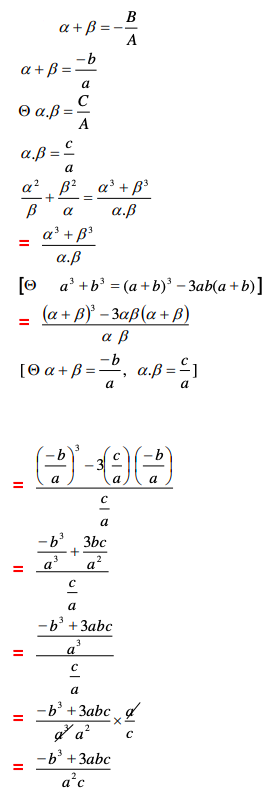OR

[ii] x2 – [11x / 4] + [15 / 8] = 0

8x2 – 22x + 15 / 8 = 0

8x2 – 22x + 15 = 0

8x2 – 12x − 10x + 15 = 0

4x (2x – 3) – 5 (2x – 3) = 0

(2x – 3) (4x – 5) = 0

2x – 3 = 0 Or 4x – 5 = 0

x = 3 / 2 or x = 5 / 4

Question 19: [i] A building is surmounted by a flag, from a point on the ground 20 meters away from the foot of a building, the angle of elevation of the top of the building and flag are 45° and 60°. Find the height of the building and length of the flag. 4M

OR

[ii] From the top of a 50-meter high hill, the angle of depression of the top and the bottom of a tower is 30° and 45°. Find the height of the tower.

Solution:

[i]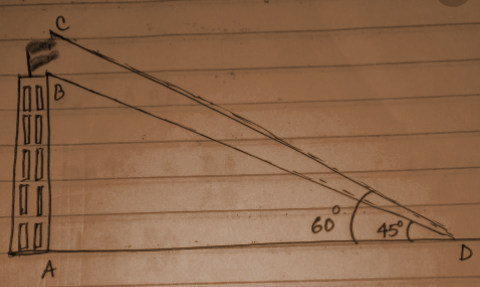tan 45o = 20 / x

x = 20m = height of the building

tan 60o = h + 20 / x

√3 = h + 20 / 20

h = 20 (√3 − 1)

Height of the flag = 14.28m

OR

[ii]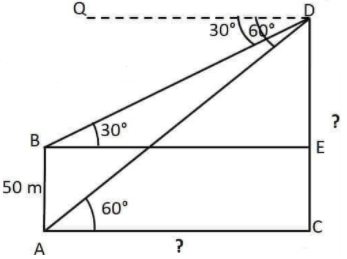To find the height of the tower CD

Draw BE parallel to AC and DQ.

Lines DQ and BE are parallel, BD is the transversal.

∠DBE = ∠QDB [alternate angles]

∠DBE = 30o

Lines DQ and AC are parallel, AD is the transversal.

∠DAC = ∠QDA [alternate angles]

∠DAC = 60o

AC and BE are parallel lines.

AC = BE

Similarly, AB and CE are also parallel lines.

CD = AB

CD = 50m

Since DC is perpendicular to AC, ∠DEB = ∠DCA = 90o.

tan 30o = DE / BE

1 / √3 = DE / BE

BE = √3 DE — (1)

tan 60o = DC / AC

3 = DC / AC

AC = DC / √3 — (2)

From (1) and (2),

√3 DE = DC / √3

DE √3 * √3 = DC

3DE = DC

3DE = DE + EC

3DE – DE = EC

2DE = EC

DE = 50 / 2

DE = 25m

Height of the tower = DC

= DE + EC

= 25 + 50

= 75m

Question 20: [i] Find the length of arc and area of the sectors, if the angles subtended at the centre and the radii are 120° and 2.1cm respectively. 4M

OR

[ii] The radius and height of a cylinder are in the ratio of 2:3. If the volume of the cylinder is 1617 cm3, then find its whole surface area.

Solution:

[i] l = [θ / 360o] * 2𝛑r

= [120 / 360] * 2 (22 / 7) * (2.1)

= [0.333] * 13.195

= 4.4cm

Area of the sector = ½ r² θ

= (0.5) * (2.1)2 * (120o)

= 264.6 cm2

OR

[ii] Let the radius and base of the cylinder be 2x and 3x cm respectively.

Volume of cylinder = πr2h = 1617 cm3

[22 / 7] * (2x)2 * 3x = 1617

x3 = (1617 * 7) / 22 * 4 * 3 = 343 / 8

Take the cube root of both sides.

x = 7 / 2 = 3.5

So, radius = 2 * 3.5 = 7 cm and the height = 3.5 * 3 = 10.5 cm.

Total Surface area of cylinder = 2πr (r + h)

= 2 * [22 / 7] * 7 (7 + 10.5)

= 2 * 22 * 17.5

= 770 cm2

Question 21: [i] Three solid spheres having the diameters 2 centimetres, 12 centimetres and 16 centimetres respectively are melted to form a sphere. Find the radius of the sphere. 4M

OR

[ii] An iron sphere of diameter 6 centimetres is melted and drawn into a cylindrical wire. If the diameter of the wire is 0.2 centimetre, then find the length of the wire.

Solution:

[i] Let the radius of the solid sphere be ‘R’ cm.

[4 / 3]πR3 = [4 / 3]π[2 / 2]3 + [4 / 3]π[12 / 2]3 + [4 / 3]π[16 / 2]3

R3 = 1 + 216 + 512

R3 = 729 = 93

R = 9cm

The radius of the sphere is 9cm.

OR

[ii] For sphere, d = 6cm, radius = 6 / 2 = 3cm

For cylindrical wire, d = 0.2cm, radius = 0.2 / 2 = 0.1cm

Volume of cylindrical wire = Volume of the sphere

πr2h = [4 / 3]πr3

π * 0.12 * h = [4 / 3] * π * 33

h = 113.01 / 0.0314

h = 3600cm or h = 36m

Question 22: [i] Find all other zeros of polynomial f (x) = x3 + 13x2 + 32x + 20, if one of the zeros is -2. 5M

OR

[ii] Factorise: a2 (b + c) + b2 (c + a) + c2 (a + b) + 2abc.

Solution:

[i] f (x) = x3 + 13x2 + 32x + 20

It is given that -2 is a zero of the function. It means (x + 2) is a factor of a given polynomial.

Divide f (x) by (x + 2), to find the remaining factor.

x3 + 13x2 + 32x + 20 / [x + 2] = x2 + 11x + 10

The function can be written as

f (x) = (x + 2) (x2 + 11x + 10)

f (x) = (x + 2) (x2 + 10x + x + 10)

f (x) = (x + 2) (x (x + 10) + 1 (x + 10))

f (x) = (x + 2) (x + 10) (x + 1)

Equate f (x) = 0, to find the zeros.

(x + 2) (x + 10) (x + 1) = 0

x = -2, -10, -1

Therefore, all zeroes of the polynomial are -10, -2 and -1.

OR

[ii] a2 (b + c) + b2 (c + a) + c2 (a + b) + 2abc

= a2 b + a2c + b2c + ab2+ c2a + bc2 + 2abc

= a (b2 + 2bc + c2) + a2 b + a2c + b2 c + bc2

= a (b + c)2 + a2 (b + c) + bc (b + c)

= (b + c) [ a2 + a (b + c) + bc]

= (b + c) (a + b) (c + a)

Question 23: [i] Solve by using formula 10y2 – 11y – 6. 5M

OR

[ii] The sum of the ages of a man and his son is 45 years. Four years ago, the product of their ages was 160. Find their present ages.

Solution:

[i] 10y2 – 11y – 6 = 0

a = 10, b = -11, c = -6

y = -b ± √b2 – 4ac / [2a]

= 11 ± √121 – 4 * (10) * (-6) / [2 * 10]

= 11 ± √121 + 240 / 

= 11 ± 19 / 20

y = 11 + 19 / 

y = 30 / 20

y = 3 / 2 and

y = 11 – 19 / 2

y = -8 / 2

y = -4

OR

[ii] Let the present age of father = x years and the present age of son = y years

According to question:

x + y = 45 …(1)

Again, 4 years ago, the product of their ages was 160, therefore,

(Age of man 4 years ago) × (Age of son 4 years ago) = 160

(x – 4) × (y – 4) = 160

⇒ (45 – y – 4) × (y – 4) = 160 [Using (1)]

⇒ 45y – 180 – y2 + 4y – 4y + 16 = 160

⇒ – y2 + 45y – 324 = 0

⇒ y2 – 36y – 9y + 324 = 0

⇒ y (y – 36) –9 (y – 36) = 0

⇒ (y – 36) (y – 9) = 0

⇒ y = 36 or y = 9

For y = 36, x = 45 – 36 = 9 which is not possible.

For y = 9, x = 45 – 9 = 36

Question 24: [i] Find the difference between compound interest and simple interest for Rs, 2000 at the rate of 5% per annum interest for 3 years. 5M

OR

[ii] A watch is sold for Rs. 960 cash or for Rs. 480 cash down payment and two monthly instalments of Rs. 245 each. Find the rate of interest charged under the instalment plan.

Solution:

[i] Principal = Rs. 2000

Rate of interest = R = 5%

Time = 3 years

SI = [PTR] / 100

= [2000 * 5 * 3] / 100

= 300

CI = Amount – Principal

A = P [1 + R]t

A = 2000 [1 + 0.05]3

A = Rs. 2315.25

CI = Amount – Principal

CI = 2315.25 – 2000

CI = 315.25

Difference between SI and CI = 315.25 – 300 = Rs. 15.25.

OR

[ii] Cash price of watch = Rs. 960

Cash down payment = Rs. 480

Balance due = 960 − 480 = Rs. 480

Total payment = 245 × 2 = Rs. 490

Total interest paid in instalment

= Rs. 490 − Rs. 480

= Rs. 10

Principal for 1st month = Rs. 480

Principal for 2nd month = Rs. 480 − 245

= Rs. 235

Total principal for 1 month = Rs.480 + Rs. 235

= Rs. 715

∴ Rate of interest in the instalment

= [Interest * 100] / [Principal * time]

= [10 × 100] / [715 × (1 / 12)]

= 1000 × 12 / 715

= 16.78%

Question 25: [i] Construct the incircle of the equilateral triangle whose one side is 8 centimetre. Measure its radius. 5M

OR

[ii] Construct a ∆ABC, similar to a given ∆ABC whose sides are in the ratio 7:5.

Solution:

[i]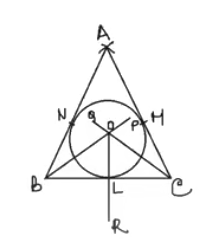Construction of △ABC –

(1) Draw a line segment BC=8 cm.

(2) Draw an arc of radius =8 cm taking B as the centre.

(3) Draw another arc of radius =8 cm taking B as the centre.

(4) Draw another arc of radius =8 cm. taking C as the centre with intersects the previous arc at a point A.

(5) Join AB and AC.

Thus the require △ABC is constructed.

Construction of the incircle of △ABC.

(1) Draw BP and CQ the bisectors of angles ∠B and ∠C respectively and which intersect each other at point O.

(2) Draw OR⊥BC which intersects BC at L.

(3) Taking O as the centre and OL as radius draw a circle which touches the sides AB, BC and CA at points N, L and M respectively.

(4) Measure the radius OL which is 2.3 cm.

Thus the required incircle is constructed and its radius = 2.3 cm.

OR

[ii]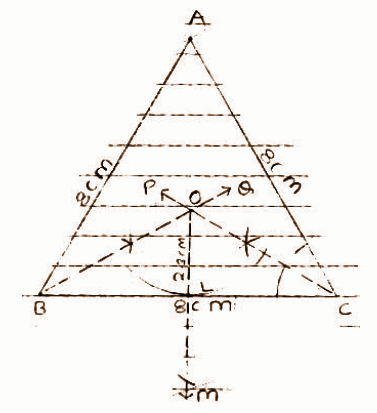Question 26: [i] Simplify the expression (1 + cot θ – cosec θ) (1 + tanθ + sec θ).

5M

OR

[ii] Prove it without using the table: sec 37o / cosec 53o + sin 42o / cos 48o = 2.

Solution:

[i] The given expression isθ

(1 + cot θ – cosec θ) (1 + tanθ + sec θ)

Simplifying the given expression,

(1 + cot θ – cosec θ) (1 + tan θ + sec θ)

= (1 + cos θ / sin θ – 1/ sin θ) (1 + sin θ / cos θ + 1 / cos θ)

= (sin θ + cos θ – 1) / sin θ * (cos θ + sin θ + 1) / cos θ

= ((sin θ + cos θ – 1) (cos θ + sin θ + 1)) / (sin θ cos θ)

= ({(sin θ + cos θ) – 1}{(sin θ + cos θ) + 1}) / (sin θ cos θ)

= ((sin θ + cos θ)2 – (1)2) / (sin θ cos θ)

= ((sin θ + cos θ)2 – (1)2) / (sin θ cos θ)

= ((sin2 θ + cos2 θ + 2 sin θ cos θ) – 1) / (sin θ cos θ)

= ((sin2θ + cos2θ) + 2 sin θ cos θ – 1) / (sin θ cos θ)

= (1 + 2 sin θ cosθ – 1) / (sinθ cos θ)

= (2 sin θ cos θ) / (sin θ cos θ)

OR

[ii] LHS = sec 37o / cosec 53o + sin 42o / cos 48o

= sec (90 – 53) / cosec 53 + sin (90 – 48) / cos 48

= cosec 53 / cosec 53 + cos 48 / cos 48

= 1 + 1

= 2